• OFDM 原理框图OFDM.pdf发送方大法师法大法师法
• ## OFDM原理

千次阅读 2016-06-08 14:11:58
章节一：时域上的OFDM 　OFDM的"O"代表着"正交"，那么就先说说正交吧。 　首先说说最简单的情况，sin(t)和sin(2t)是正交的【证明：sin(t)·sin(2t)在区间[0,2π]上的积分为0】，而正弦函数又是波的最直观描述，因此...

章节一：时域上的OFDM
OFDM的"O"代表着"正交"，那么就先说说正交吧。
首先说说最简单的情况，sin(t)和sin(2t)是正交的【证明：sin(t)·sin(2t)在区间[0,2π]上的积分为0】，而正弦函数又是波的最直观描述，因此我们就以此作为介入点。既然本文说的是图示，那么我们就用图形的方式来先理解一下正交性。【你如果能从向量空间的角度，高屋建瓴的看待这个问题的话，你也就不是"小白"了，R U?】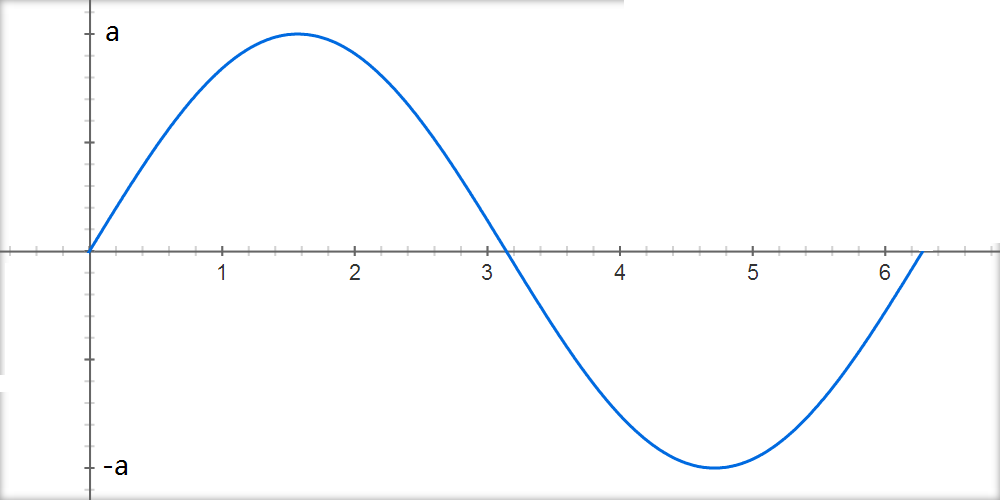图一：发送a信号的sin(t)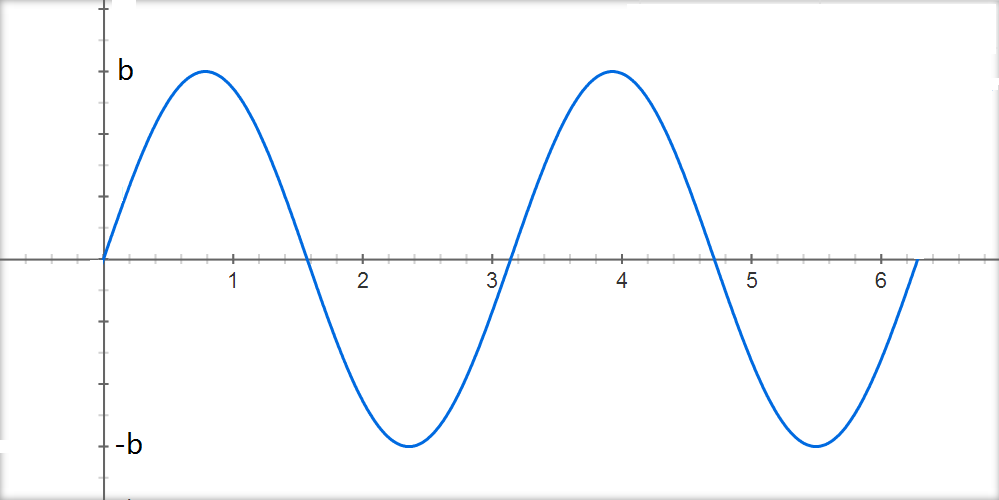图二：发送b信号的sin(2t)【注意：在区间[0,2π]内发送了两个完整波形】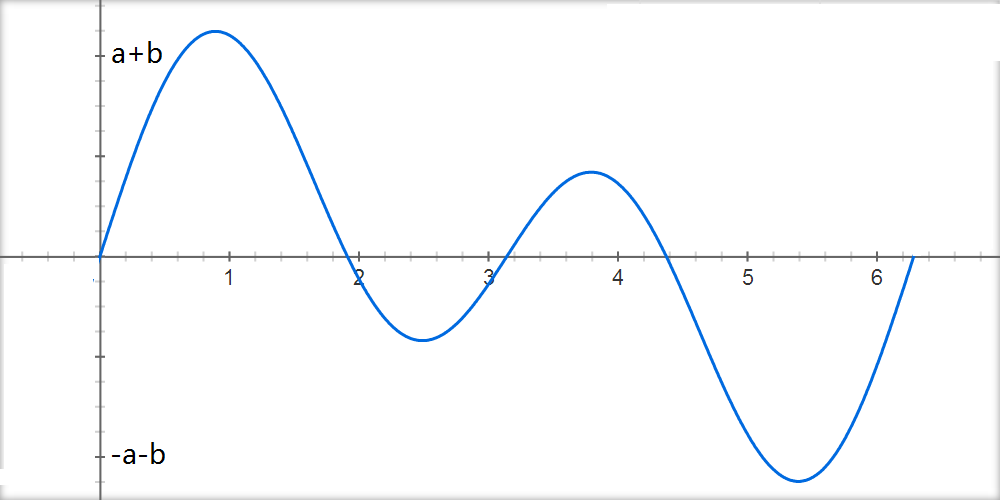图三：发送在无线空间的叠加信号a·sin(t)+b·sin(2t)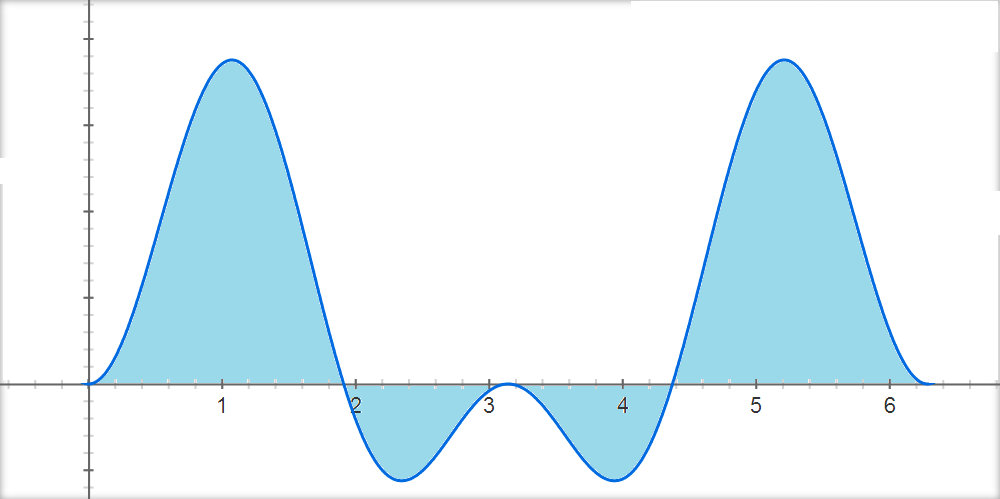图四：接收信号乘sin(t)，积分解码出a信号。【如前文所述，传送b信号的sin(2t)项，在积分后为0】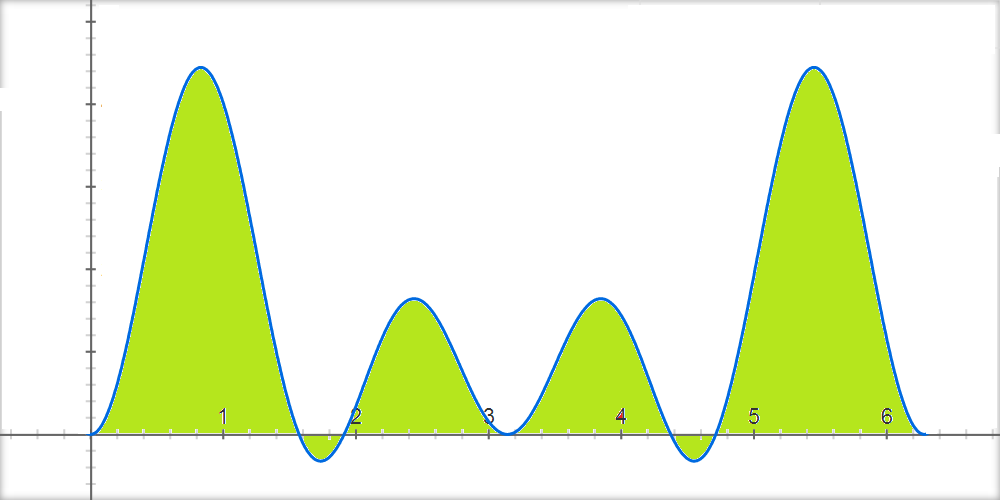图五：接收信号乘sin(2t)，积分解码出b信号。【如前文所述，传送a信号的sin(t)项，在积分后为0】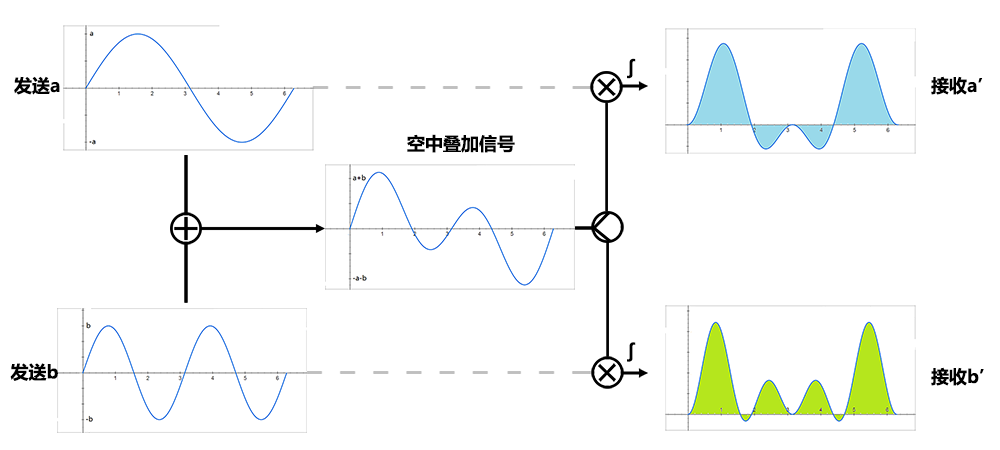图六：流程图

到了这里，也许你会出现两种状态：
一种是：啊，原来是这样，我懂了。
一种是：啊，怎么会这样，我完全无法想象。这里要说的是，你根本用不着去想象（visualize）。数学中是如此定义正交的，数学证明了它们的正交性，那么他们就是正交的，【他们就可以互不干扰的承载各自的信息】。选取sin(t)和sin(2t)作为例子，正是因为它们是介于直观和抽象的过渡地带，趟过去吧。

上面的图示虽然简单，但是却是所有复杂的基础。
1.1 下一步，将sin(t)和sin(2t)扩展到更多的子载波序列{sin(2π·Δf·t)，sin(2π·Δf·2t)，sin(2π·Δf·3t),...,sin(2π·Δf·kt)} (例如k=16，256，1024等)，应该是很好理解的事情。其中，2π是常量；Δf是事先选好的载频间隔，也是常量。1t,2t,3t,...,kt保证了正弦波序列的正交性。
1.2 再下一步，将cos(t)也引入。容易证明，cos(t)与sin(t)是正交的，也与整个sin(kt)的正交族相正交。同样，cos(kt)也与整个sin(kt)的正交族相正交。因此发射序列扩展到{sin(2π·Δf·t),sin(2π·Δf·2t),sin(2π·Δf·3t),...,sin(2π·Δf·kt),cos(2π·Δf·t),cos(2π·Δf·2t),cos(2π·Δf·3t),...,cos(2π·Δf·kt)}也就顺理成章了。
1.3 经过前两步的扩充，选好了2组正交序列sin(kt)和cos(kt)，这只是传输的"介质"。真正要传输的信息还需要调制在这些载波上，即sin(t),sin(2t),...,sin(kt)分别幅度调制a1,a2,...,ak信号,cos(t),cos(2t),...,cos(kt)分别幅度调制b1,b2,...,bk信号。这2n组互相正交的信号同时发送出去，在空间上会叠加出怎样的波形呢？做简单的加法如下：
f(t) = a1·sin(2π·Δf·t) +         a2·sin(2π·Δf·2t) +         a3·sin(2π·Δf·3t) +         ...        ak·sin(2π·Δf·kt) +         b1·cos(2π·Δf·t) +         b2·cos(2π·Δf·2t) +         b3·cos(2π·Δf·3t) +         ...        bk·cos(2π·Δf·kt) +       = ∑ak·sin(2π·Δf·kt) + ∑bk·cos(2π·Δf·kt) 【公式1-1：实数的表达】
为了方便进行数学处理，上式有复数表达形式如下： f(t) = ∑Fk·e(j·2π·Δf·kt) 【公式1-2：复数的表达，这编辑器找不到上角标...不过，你应该看得懂的】
上面的公式可以这样看：每个子载波序列都在发送自己的信号，互相交叠在空中，最终在接收端看到的信号就是f(t)。接收端收到杂糅信号f(t)后，再在每个子载波上分别作相乘后积分的操作，就可以取出每个子载波分别承载的信号了。
然后，多看看公式1-1和公式1-2！！！发现咯？这就是傅里叶级数嘛。如果将t离散化，那么就是离散傅立叶变换。所以才有OFDM以FFT来实现的故事。将在下面的章节进行更多的描述。
遵循古老的传统，F表示频域，f表示时域，所以可以从公式1-2中看出，每个子载波上面调制的幅度，就是频域信息。类似的说法是：OFDM传输的是频域信号。这种说法有些别扭，但是很多教程或文章会使用这样的说明方式，就看读者如何理解了。如果纯粹从公式或者子载波来看，这种说法其实也是很直接的阐述了。
上面1.1-1.3的扩展，可如下图所示：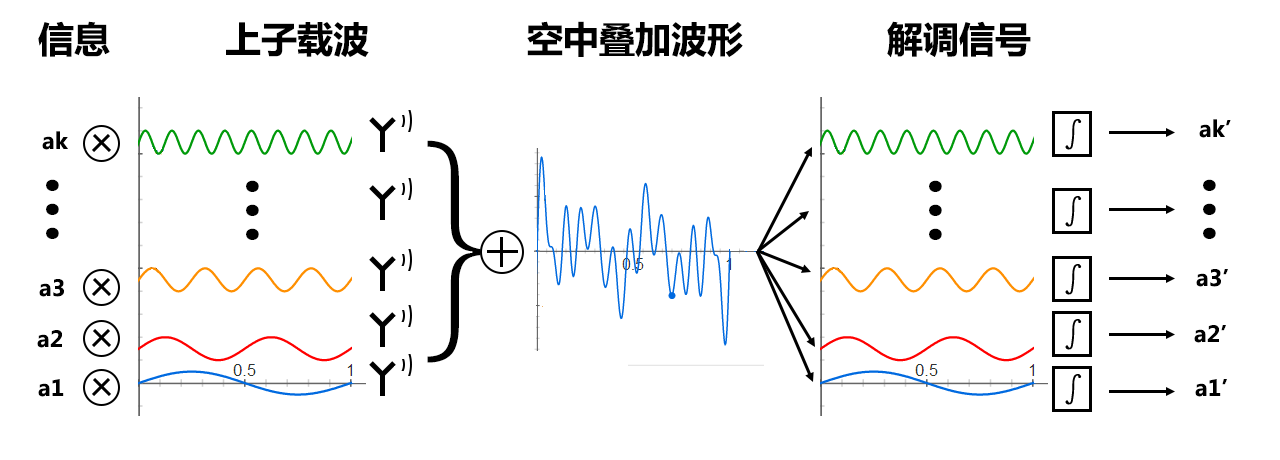图七：时域上的OFDM系统图   　　1.4 还有这一步吗？其实是有的。"小白"你可以先想想，想不到的话先往下看，因为这需要在频域中考量，所以我写在后面了。【也可参考】

将上述的时域分析配上LTE的实现，有如下情况： 　　【注1：本段描述需要有LTE物理层的基本知识，如果看不明白，请暂时跳过，看完整篇文章后再回看】 　　【注2：LTE并非时域的实现，下面仅仅是套用LTE的参数，做一个参考分析】
子载波的间隔Δf=15kHz，一个OFDM symbol的发送时间是66.7us，可以发现，15kHz*66.67us=1，即基带上一个OFDM symbol的发送时间正好发送一个一次谐波的完整波形。对于10M的LTE系统，采用的是1024个子载波，但是只有中间600个（不含最中间的直流）子载波被用于传送数据。在一个OFDM symbol的时间内（即66.67us)，靠近中间的两个一次谐波传输一个完整波形，再靠外一点的两个二次谐波传输两个完整波形，以此类推至最外面的两个300次谐波传输了300个完整的波形。在这66.67us内，600个子载波互相正交，其上分别承载了600个复数信号。
上面的说法有点啰嗦，不如图示来得直观。本来准备再画一图的，不过一来上面已经有了类似的图，实是大同小异；二来，600个子载波，也太多了点。。。
负频率：负频率的说法大抵是引入复数后，计算中的产物。sin(kt)和cos(kt)两组信号提供了一个最小完备空间希尔伯特向量空间的基底。在表达为复数信号的时候，一个复数实际上传输了两个数据，分别承载在sin核cos载波上。 　　OK，说到这里，从时域上面来看OFDM，其实是相当简洁明快讨人喜欢的。不过，一个系统若要从时域上来实现OFDM，难度太大，时延和频偏都会严重破坏子载波的正交性，从而影响系统性能。这点在各种教材文章中都会有提及，我就不赘述了。
下面将转入频域来描述OFDM，由于频域不甚直观，的确会稍稍让人费解。不过只要时刻想着时域子载波间的叠加，一切都会好起来。

章节二：频域上的OFDM

第一章节时域上的讨论开始于OFDM中的"O"；本章节频域上我们从"FDM"开始。 　　先图例一个常规FDM的系统图：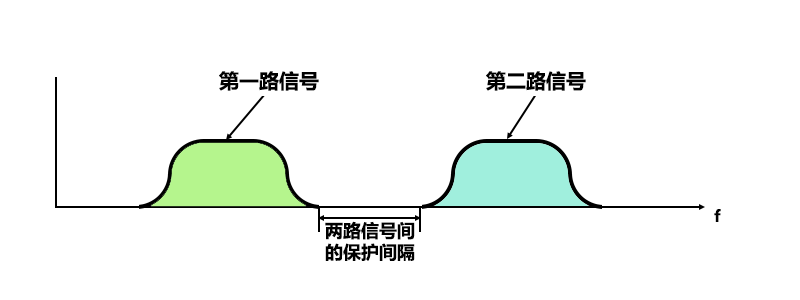图11：常规FDM，两路信号频谱之间有间隔，互相不干扰  　　为了更好的利用系统带宽，子载波的间距可以尽量靠近些。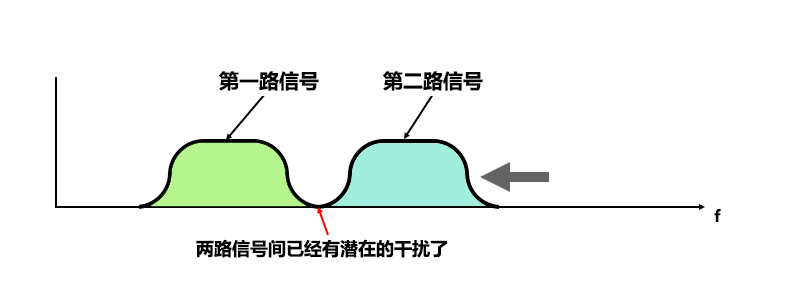图12：靠得很近的FDM，实际中考虑到硬件实现，解调第一路信号时，已经很难完全去除第二路信号的影响了（电路的实现毕竟不能像剪刀裁纸一样利落），两路信号互相之间可能已经产生干扰了  　　还能再近些吗？可以的。这就是OFDM的来历啊，近到完全等同于奈奎斯特带宽（后面有详述），使频带的利用率达到了理论上的最大值。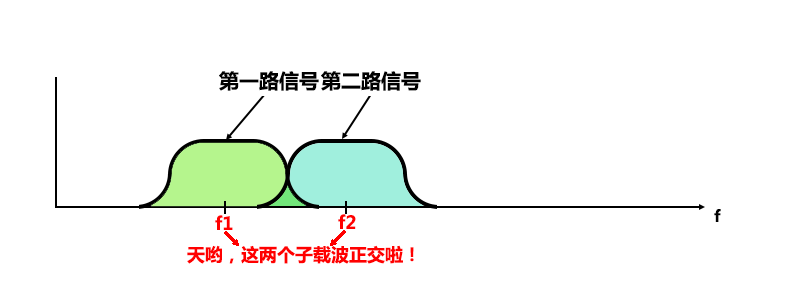图13：继续靠近，间隔频率互相正交，因此频谱虽然有重叠，但是仍然是没有互相干扰的。神奇的OFDM  　　上面三个图的确有点小儿科，不知道"小白"是不是已经在心里呐喊：这谁不知道呀！不过我在这里花时间画了三张图，总还是有所考量的： a. 作为上一个章节和本章节之间的补充和连接，说明一下OFDM在频域上面的表现，亦即OFDM的本源来历。 b. 引导思考：信号的带宽是多少？ c. 引导思考：OFDM正交频谱叠加部分到底有多宽呢？结合1.4，先想想，再往下看，会更好。
再次回到正轨，请回看第一节中的图一至图六等时域波形图，图示了在时域上，波形的调制，叠加接收，以及最终的解码。让我们看看图一至图三中的每个步骤在频域上是如何表现的。
首先来看sin(t)。"小白"呀"小白"，你且说说sin(t)的频谱是啥呀？"小白"弱弱的说：是一个冲激。是的，sin(t)是个单一的正弦波，代表着单一的频率，所以其频谱自然是一个冲激。不过其实图一中所示的sin(t)并不是真正的sin(t)，而只是限定在[0,2π]之内的一小段。无限长度的信号被限制在一小截时间之内，【就好比从一个完整的人身上逮下一根头发，然后把整个人都丢掉，以发代人】其频谱也不再是一个冲激了。
对限制在[0,2π]内的sin(t)信号，相当于无限长的sin(t)信号乘以一个[0,2π]上的门信号（矩形脉冲），其频谱为两者频谱的卷积。sin(t)的频谱为冲激，门信号的频谱为sinc信号（即sin(x)/x信号）。冲激信号卷积sinc信号，相当于对sinc信号的搬移。所以分析到这里，可以得出图一的时域波形其对应的频谱如下：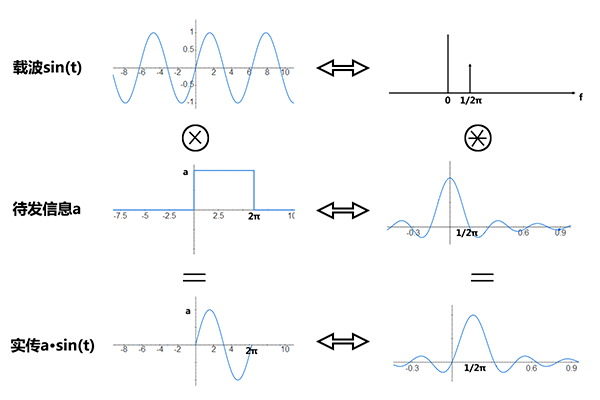图21：限定在[0,2π]内的a·sin(t)信号的频谱，即以sin(t)为载波的调制信号的频谱  　　sin(2t)的频谱分析基本相同。需要注意的是，由于正交区间为[0,2π]，因此sin(2t)在相同的时间内发送了两个完整波形。相同的门函数保证了两个函数的频谱形状相同，只是频谱被搬移的位置变了：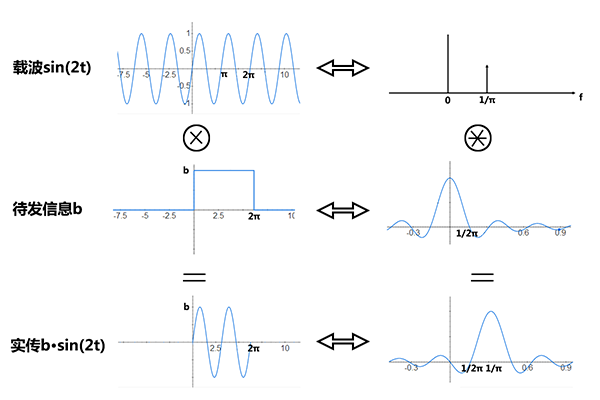图22：限定在[0,2π]内的b·sin(2t)信号的频谱，即以sin(2t)为载波的调制信号的频谱  　　将sin(t)和sin(2t)所传信号的频谱叠加在一起，如下：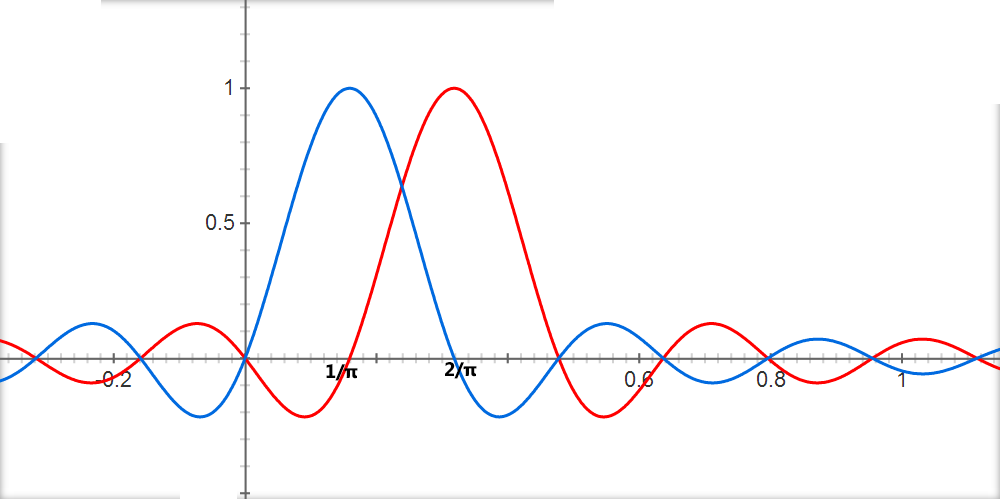图23：a·sin(t)+b·sin(2t)信号的频谱   　　图23和图13，均是频域上两个正交子载波的频谱图。比一下，发现了吗？不太一样！
是的，想必你已经想起来了，这是因为基带信号在传输前，一般会通过脉冲成型滤波器的结果。比如使用"升余弦滚降滤波器"后，图23所示的信号就会被修理成图13所示的信号了。这样可以有效的限制带宽外部的信号，在保证本路信号没有码间串扰的情况下，既能最大限度的利用带宽，又能减少子载波间的各路信号的相互干扰。这也是1.4中没有提及的，更多的可参考
贴士：脉冲成型滤波器作用于频域，可以"看作"时域中的每个码元都是以类似sinc信号发出的。没必要纠结于发送端码元的时域波形，只需要知道在接收端通过合适的采样就可以无失真的恢复信号就OK咯。
这里用到的是奈奎斯特第一准则，在下面的框框内会稍作描述：

奈奎斯特第一准则请自行google，这里说说其推论：码元速率为1/T(即每个码元的传输时长为T)，进行无码间串扰传输时，所需的最小带宽称为奈奎斯特带宽。 　　对于理想低通信道，奈奎斯特带宽W = 1/(2T) 　　对于理想带通信道，奈奎斯特带宽W = 1/T  　　在下面的图31中，可以看出信号的实际带宽B是要大于奈奎斯特带宽W（低通的1/(2T)或者带通的1/T）的，这就是理想和现实的距离。 　　补充说明：本文提到的"带宽"，也即约定俗成的带宽理解方式，指的是信号频谱中>=0的部分。在从低通到带通的搬移过程中，因为将原信号负频率部分也移出来了（也可理解为同乘e(j2πfct) + e(-j2πfct)的结果，见参考）【注：没有上角标和下角标的编辑器，真不爽。不过，你应该看得懂的】，所以带宽翻倍了。如下图所示：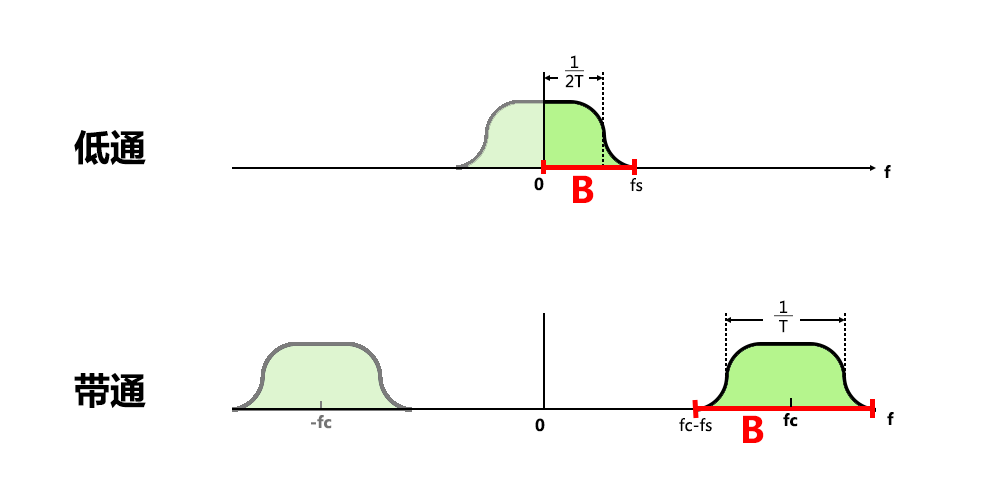图31：内涵丰富的图，请参看上面和下面的说明文字

上面专门用框框列出奈奎斯特第一准则，还有一个重要目的就是说明下频带利用率的问题。频带利用率是码元速率1/T和带宽B(或者W)的比值。
理想情况下，低通信道频带利用率为2Baud/Hz；带通信道频带利用率在传输实数信号时为1Baud/Hz，传送复数信号时为2Baud/Hz（负频率和正频率都独立携带信号）。由于讨论低通信道时往往考虑的是实数信号，而讨论带通信道时通常考虑的是复数信号，因此可以简单认为：理想情况下，信道的频带利用率为2Baud/Hz。
实际情况下，因为实际带宽B要大于奈奎斯特带宽W，所以实际FDM系统的频带利用率会低于理想情况。
【说到这里，终于可以图穷匕见了】而OFDM的子载波间隔最低能达到奈奎斯特带宽，也就是说（在不考虑最旁边的两个子载波情况下），OFDM达到了理想信道的频带利用率。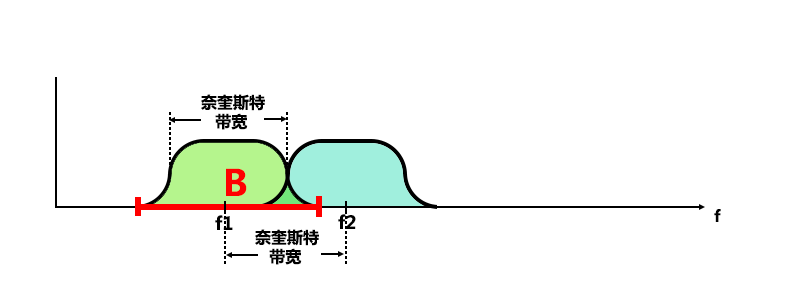图32：OFDM正交子载波，载频间距为奈奎斯特带宽，保证了最大的频带利用率   　　将上述的频域分析配上LTE的实现，有如下情况： 　　【注：本段描述需要有LTE物理层的基本知识】
子载波的间隔Δf=15kHz，一个OFDM symbol的发送时间是66.7us。在10MHz信道上，1ms的子帧共传输14个OFDM symbol【不是15个，留空给CP了】，每一个OFDM symbol携带600个复数信息，因此： 　　1. 从整个系统来看，波特率为600*14*2/1ms = 16.8MBaud，占据带宽10MHz，因此带宽利用率为16.8MBaud/10MHz = 1.68Baud/Hz，接近2Baud/Hz的理想情况。【注：一是CP占用了每个OFDM Symbol约1/15的资源，二是10MHz的频带并不是满打满算的用于传输数据，其边界频带需要留空以减少与邻近信道的干扰】 　　2. 单从OFDM一个symbol来看，波特率为600*2/66.7us = 18MBaud，占据带宽600*15kHz=9MHz【不考虑边界子载波带外问题】，因此其带宽利用率为18MBaud/9MHz=2Baud/Hz，符合上面的讨论。 　　附：5M带宽的WCDMA的chip rate = 3.84M/s，即码率为3.84M*2 = 7.68MBaud，带宽5M，所以带宽利用率为7.68MBaud/5MHz = 1.536Baud/Hz，略逊于LTE的1.68Baud/Hz【注：WCDMA的脉冲成型采用滚降系数为0.22的升余弦滤波器，奈奎斯特带宽为3.84M】

章节三：用IFFT实现OFDM
其实前两章，我已经将自己的理解尽数表达了：第一节是从时域上来说子载波正交的原理；第二节是从频域上来解释子载波正交后，达到理想频带利用率的特性。想来，虽然前两章写得较长【没预料到会写这么长的...太长了没人看...】，但是应该还是很简单、清晰、易懂的。 　　不过"小白"的卡壳，似乎并不在于最基本的正交原理和频带利用率上，反而是IFFT变换中，充斥的各种时域频域角色变换让其眼花缭乱。
个人觉得要理解IFFT实现OFDM，最好的办法还是看公式。比如第一章节中的公式1-1和公式1-2，配上时域波形图的叠加，不要太好理解哟。当然，这里的IFFT需要将时域离散化，因此公式IFFT ≈ IDFT -->
fn = 1/N·∑Fk·e(j·2π·k·n/N) 【公式3-1，n为时域离散后的序号，N为总的IFFT个数，n∈[1,N]】
关于公式3-1的理解方法，可以是这样的。其中一种理解方式是联系第一章节的公式1-2：可以发现公式3-1等号右侧所表达的物理意义和公式1-2是相同的，均代表了不同子载波e(j·2π·k·n/N)发送各自的信号Fk，然后在时域上的叠加形成fn，只不过现在叠加出来的时域不是连续波形，而是离散的时序抽样点。
另一种更容易，更可爱的理解方式是：在一个OFDM symbol的时长T内，用N个子载波各自发送一个信号F(k)（k∈[1,N]），等效于直接在时域上连续发送fn（n∈[1,N]）N个信号，每个信号发送T/N的时长。
在IFFT实现OFDM中，发送端添加了IFFT模块、接收端添加了FFT模块。IFFT模块的功能相当于说：别麻烦发送N个子载波信号了，我直接算出你们在空中会叠加成啥样子吧；FFT模块的功能相当于说：别用老式的积分方法来去除其余的正交子载波了，我帮你一次把N个携带信号全算出来吧。就是这样，IFFT实现OFDM的系统用"数学的方法"，在发送端计算信号的叠加波形，在接收端去除正交子载波，从而大大简化了系统的复杂度。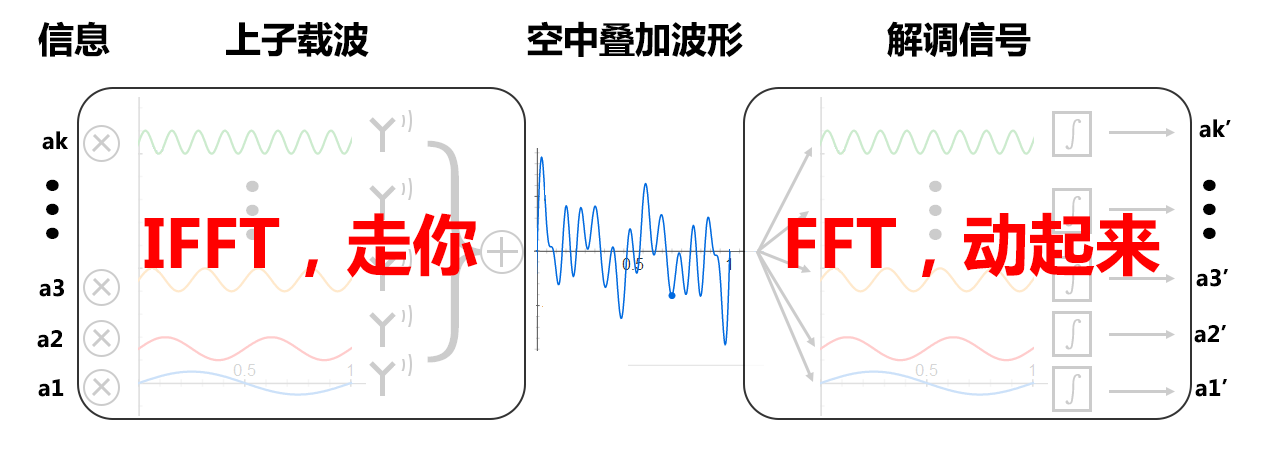图八：用IFFT实现OFDM。请自行对比图七   　　最后说一句："小白"乃"白富美"之"白"，非"一穷二白"之"白"也。 　　好吧，该结束了。再写得长了更没人看了。

补充章节：从频谱上来看正交性

本文最开始发表时是没有这一段的，因为原文已然十分自恰，已将OFDM的原理说的非常清楚到位了。然而，这一段的内容却是别的文章中讲解OFDM时经常出现的桥段，因此觉得还是有必要补充陈述一下自己的观点。

【注：本小节为补充章节，与本文逻辑没有必然联系，可直接略过。】

从正文章节中，可以发现
作者的思路：从时域角度讲解子载波的正交性；从频域角度讲解OFDM的频带利用率。作者觉得这是最容易理解OFDM原理的方式。但是教材中、网络上，还有一种非常主流的讲解方式：
从频域上“直观的”看待子载波的正交性。比如下面这个图：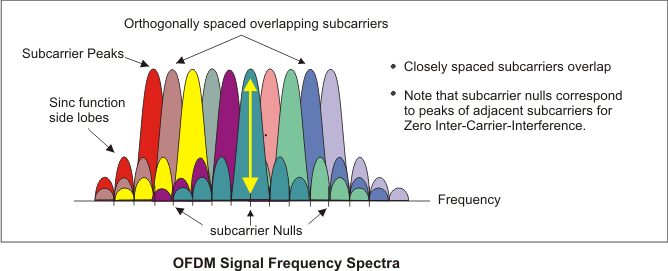图51：从OFDM频谱看待正交性（
本图来自网络，比我画的图好些，还有文字说明）

这种观点的说法是：
在每个子载波的抽样点上，其它的子载波信号抽样值均为0（即上图中的subcarrier Nulls对应某个子载波的Subcarrier Peak）。这种说法在图示上有非常醒目的直观效果，所以是各教材讲义中的常客，但是至少从作者的角度来看，
这种说法在涉及到后面的解调信号时，将变得非常难以理解和说明。所以本文最开始的版本中是没打算写本小节的。

如果你看到这里，觉得这种说法正中下怀，那么恭喜你。

如果你看到这里，觉得这种说法已经让你的脑袋成了浆糊，那么可以回顾第一章节：时域上的正交性，然后继续阅读下面部分以解毒。

时域上的正交性和频域上的正交性之间的关系该如何联系起来呢？回顾前面提到sin(t)和sin(2t)是正交的【证明：sin(t)·sin(2t)在区间[0,2π]上的积分为0】，推广到更一般的情况是：{sin(2π·Δf·t)，sin(2π·Δf·2t)，sin(2π·Δf·3t),...,sin(2π·Δf·kt)}在区间[0, 1/Δf]上正交（注：教材上一般写为u(t)在[-T/2,T/2]区间上怎么怎么着，本文就用不着那么学术了）。可以看出，这里有一个关键的参数Δf：它既是频域上子载波的间距，又确定了时域上的信号传输时间。回顾时域频域转换图：图52：同前面的图21，时域波形和频域的转换

联系上图的时频转换，可以发现
Δf既确定了子载波本身(即上图中第一排的两个图)，又确定了待发信号的传输时间(即上图中第二排的两个图中信号的宽度)，从而决定了信号频谱的主瓣宽度以及旁瓣为0的位置。这也意味着，OFDM系统中一旦选定了子载波间隔，时域上的正交性以及频域上的正交性也就顺理成章的联系起来了。如下图：图53：同前面的图23，两路信号的间隔Δf，保证了时域上的正交性、确定了频域上的旁瓣0点位置

其实对本作者而言，从频谱上来看待OFDM的正交性有点颠倒因果的嫌疑。按我的理解：OFDM选用的正交子载波是因，频谱中出现“其余
子载波携带信号的旁瓣0点处于当前
子载波携带信号主瓣峰值处”的
现象是果。以果推因，谬矣。

继续补充：关于负频率

留言中不少关于
负频率的疑问，已另作一文于此：
《关于负频率的物理意义》(
http://www.jianshu.com/p/5872553401f9)

参考: Wireless Communications, Andrea Goldsmith - 12.2 Multicarrier Modulation with Overlapping Subchannels

参考: Principles of Digital Communication - Gallager - 6.4.1 Double-sideband amplitude modulation


展开全文多输入多输出 正交频分复用
• OFDM 与两者的区别 FMT - Filtered Multi-tone 用滤波器实现子载波的相互正交(无干扰) ACI - adjacent chanel interference VC - Virtual carrier 作为保护带宽的外侧子载波 ODFM通信链路（发送端） 如上所示,...
[一些基础知识]
复数
在人类的发展进程中,数的定义范围是在不断扩大的,从自然数到整数(加入负整数)到有理数(加入分数)到实数(加入无理数)再到复数(加入虚数). 其中,虚数的加入使得对负数开根号有了意义,从而让根号在复数上闭环.  实际上一个复数

a

+

b

j

a+bj

表示的是一个向量. 只不过我们可以用复平面来表示这个向量, 复平面(Re-Im)很像直角坐标平面(x-y). 在复平面上,

j

j

的作用是把一个复数逆时针旋转90度. 比如本来b是一个横轴正方向上的数,逆时针旋转90后就变成纵轴正方向的数了. 进一步来看,

i

2

=

−

1

i^2=-1

也就很好理解了,因为转了180度.  注意复平面并不是直接坐标平面. 比如

y

=

a

x

2

+

b

x

+

c

y=ax^2+bx+c

. 我们都知道

y

=

0

y=0

的解就是看这个二次曲线和x轴的交点. 那么

Δ

=

b

2

−

4

a

c

>

0

\Delta=b^2-4ac>0

时, 二次曲线和x轴有两个交点, 因此有两个实数根

Δ

=

b

2

−

4

a

c

=

0

\Delta=b^2-4ac=0

时, 二次曲线和x轴有一个交点(顶点), 因此有一个实数根

Δ

=

b

2

−

4

a

c

<

0

\Delta=b^2-4ac<0

时, 二次曲线和x轴没有交点, 因此没有实数根 但是其实第三种情况是有两个复数根的,比如

x

2

+

1

=

0

x^2+1=0

的根是

i

i

和

−

i

-i

. 多出两个根的原因是,我们本来是在x轴上找根,也就是说我们认为x只能取实数(实数轴和x轴重叠),但现在我们在一整个复平面上找根.  复平面上的加减是与向量加减一致的,复数的乘积是模相乘,角度相加,因为复数可以写成

s

=

a

+

b

j

=

∣

s

∣

e

j

θ

s

s=a+bj=|s|e^{j\theta_s}

的形式.

s

=

a

+

b

j

=

∣

s

∣

e

j

θ

s

s=a+bj=|s|e^{j\theta_s}

这个形式引申出很elegent的欧拉公式

e

j

θ

=

c

o

s

θ

+

j

s

i

n

θ

e^{j\theta}=cos\theta+jsin\theta

. 显然对于复平面上的单位圆来说,

c

o

s

θ

+

j

s

i

n

θ

cos\theta+jsin\theta

就是圆上一点. 那么根据欧拉公式, 其实复指数

e

j

θ

e^{j\theta}

的物理含义就是顺着正实轴逆时针在单位圆上转圈

θ

\theta

度得到的点. 实际上

e

j

=

lim

⁡

n

→

∞

(

1

+

j

n

)

n

e^{j}=\lim_{n\rightarrow\infty}(1+\frac{j}{n})^n

就是把复数 1 乘以

n

n

个

(

1

+

j

n

)

(1+\frac{j}{n})

. 根据复数乘法定义, 这相当于把复数1逆时针旋转n次,每次旋转

(

1

+

j

n

)

(1+\frac{j}{n})

360

/

(

2

π

)

360/(2\pi)

度. 综上,

e

j

e^{j}

e

j

θ

e^{j\theta}

就是把复数1旋转

θ

\theta

弧度(

θ

\theta

子载波

N

N

个正交(子)载波:

{

e

j

2

π

f

s

t

=

e

j

2

π

k

T

s

t

:

k

=

0

,

1

,

.

.

.

,

N

−

1

;

0

≤

t

≤

T

s

}

\left\{ e^{j2\pi f_s t}=e^{j2\pi \frac{k}{T_s} t}: k=0,1,...,N-1; 0\leq t \leq T_s\right\}

其中

T

s

T_s

是一个OFDM symbol的持续时长。注意，这N个载波的区别，随着

t

/

T

s

t/T_s

在连续区间

[

0

,

1

]

[0,1]

内的变化，他们的旋转速度是不同的。特别的, 当

t

/

T

s

t/T_s

从

0

0

到

1

1

, –

k

=

0

k=0

, 角度（相位）始终不变化; –

k

=

1

k=1

, 角度转一圈，从

0

0

到

2

π

2\pi

; –

k

=

2

k=2

, 角度转两圈，从

0

0

到

4

π

4\pi

; –

k

=

N

−

1

k=N-1

, 角度转

N

−

1

N-1

圈，从

0

0

到

2

(

N

−

1

)

π

2(N-1)\pi

;  所谓的

N

N

个正交载波是指它们两两正交。其中，第i-th载波和第j-th载波正交的定义是

1

T

s

∫

0

T

s

e

j

2

π

i

T

s

t

[

e

j

2

π

j

T

s

t

]

∗

d

t

=

δ

(

i

−

j

)

\frac{1}{T_s}\int_0^{T_s}e^{j2\pi\frac{i}{T_s}t}\left[e^{j2\pi\frac{j}{T_s}t}\right]^*dt=\delta(i-j)

这是连续函数在

[

0

,

T

s

]

[0,T_s]

上的积分，如果拆成实部虚部分别积分，会发现当

i

≠

j

i\neq j

时，实部虚部均是正交的。  上文中，我们考虑了在一段时间长度

[

0

,

T

s

]

[0,T_s]

，上面有

N

N

个子载波，且两两正交。现在我们考虑对这一段时间长度均匀采样。采样速度我们选为

T

s

/

N

T_s/N

，那么采样时间为

{

n

T

s

/

N

+

τ

:

n

=

0

,

1

,

2

,

.

.

.

,

N

−

1

;

0

≤

τ

≤

T

s

/

N

}

\left\{nT_s/N+\tau:n=0,1,2,...,N-1;0\leq\tau\leq T_s/N \right\}

得到了

N

N

个采样点。我们发现，对于不同的子载波，这

N

N

个采样点构成的向量仍然是正交的。具体来说，对于第i-th载波和j-th载波仍然满足

1

N

∑

n

=

0

N

−

1

e

j

2

π

i

T

s

(

n

T

s

N

+

τ

)

e

−

j

2

π

j

T

s

(

n

T

s

N

+

τ

)

=

1

N

∑

n

=

0

N

−

1

e

j

2

π

(

i

−

j

)

n

N

e

j

2

π

i

−

j

T

s

τ

=

δ

(

i

−

j

)

\frac{1}{N}\sum_{n=0}^{N-1}e^{j2\pi\frac{i}{T_s}(\frac{nT_s}{N}+\tau)}e^{-j2\pi\frac{j}{T_s}(\frac{nT_s}{N}+\tau)} =\frac{1}{N}\sum_{n=0}^{N-1}e^{j2\pi(i-j)\frac{n}{N}}e^{j2\pi\frac{i-j}{T_s} \tau}=\delta(i-j)

注意多出来的

τ

\tau

是一个固定的phase term，每个采样点都有相同的附加phase:

i

=

j

i=j

时候全为1，

i

≠

j

i\neq j

时，所有向量全部加成了0(刚刚好转了整数圈).  特别的，上式是一理想情况，即各个子载波的

τ

\tau

都是一样的。那如果两个子载波有时延，他们的相关性会怎样尼？

1

N

∑

n

=

0

N

−

1

e

j

2

π

i

T

s

(

n

T

s

N

+

τ

)

e

−

j

2

π

j

T

s

(

n

T

s

N

+

τ

+

Δ

τ

)

=

1

N

∑

n

=

0

N

−

1

e

j

2

π

(

i

−

j

)

n

N

e

−

j

2

π

j

Δ

τ

T

s

=

e

−

j

2

π

j

Δ

τ

T

s

δ

(

i

−

j

)

\frac{1}{N}\sum_{n=0}^{N-1}e^{j2\pi\frac{i}{T_s}(\frac{nT_s}{N}+\tau)}e^{-j2\pi\frac{j}{T_s}(\frac{nT_s}{N}+\tau+\Delta\tau)} =\frac{1}{N}\sum_{n=0}^{N-1}e^{j2\pi(i-j)\frac{n}{N}}e^{-j2\pi\frac{j\Delta\tau}{T_s} }=e^{-j2\pi\frac{j\Delta\tau}{T_s}}\delta(i-j)

与上式相比

i

=

j

i=j

时模值不变但多了一个phase term，而且这个phase随着子载波频率升高而变大。但这个phase不会超过

2

π

2\pi

。点对点ODFM中，接收信号和本地载波间有一个

Δ

τ

\Delta\tau

， 且这个

Δ

τ

\Delta\tau

对所有子载波都是一样的， 那么解出来的频域信号每个符号都多出一个相位，且相位随着子载波序号变大而变大。ODFMA中，各个接收子载波和本地子载波的

Δ

τ

\Delta\tau

都不一样，因此解出来的符号上的附加相位都不一样。
注意我们传输时并不需要利用所有的子载波, 比如

N

=

16

N=16

, 但我们只用第0, 1, 2, 3这四个子载波. 他们仍然还是正交的, 而且接收端可以采用更低的采样率了. 其实这也就相当于从

N

=

16

N=16

变成

N

=

4

N=4

.
有一个问题是,

N

=

16

N=16

时候频率最高的那个子载波采样率到底够不够nyquist rate？真正的ofdm第0个子载波是不能用的 而且还有负频率. 高频时候负频率也会变正, 那么基带负频率有什么含义?
[OFDM]
单载波通信, 多载波通信和OFDM 假设我们有一段带宽

B

B

Hz和一段需要发送的modulated symbols. – 若使用传统的单载波传输方式，我们会完全利用

B

B

Hz的带宽用

B

B

Hz的速度传输这些symbol . 那么每个symbol的持续时长是

1

/

B

1/B

s. 传输

N

N

个symbols需要的时间是

N

/

B

N/B

. – 若使用多载波的传输方式，我们可以把这

B

B

Hz的带宽分成

N

N

份, 相当于有了

N

N

个子信道进行传输。实际传输时, 我们对高速的

B

B

Hz的基带短symbol先进行串并变换，变成

N

N

路信号。特别的，每一路symbol的持续时长拉长到以前的N倍变成长信号，即

N

/

B

N/B

. 这样每一路所占的带宽仅仅是以前的

1

/

N

1/N

即

B

/

N

B/N

. 这样就可以用刚刚分好的

N

N

个子信道传输每一路数据.
注意, 多载波通信相对于单载波并没有额外增益，即还是在B的带宽上用N/B的时长传输了N个symbols。但是好处在于，N个子载波单独过信道，不容易超过相干带宽引起频率选择性衰落换言之频率信道在子载波上基本上保持不变但在整个B Hz上却很容易改变. 相干带宽由最大多径时延决定, 也就是说频率选择性衰落其实是多径衰落. 当有多径时, 短symbol收到的影响更大,也就有更严重的ISI (也就需要更强大的均衡器,而多载波不需要). 我想知道, ISI在频率上到底是什么样的衰落?有什么表现?
– OFDM 与两者的区别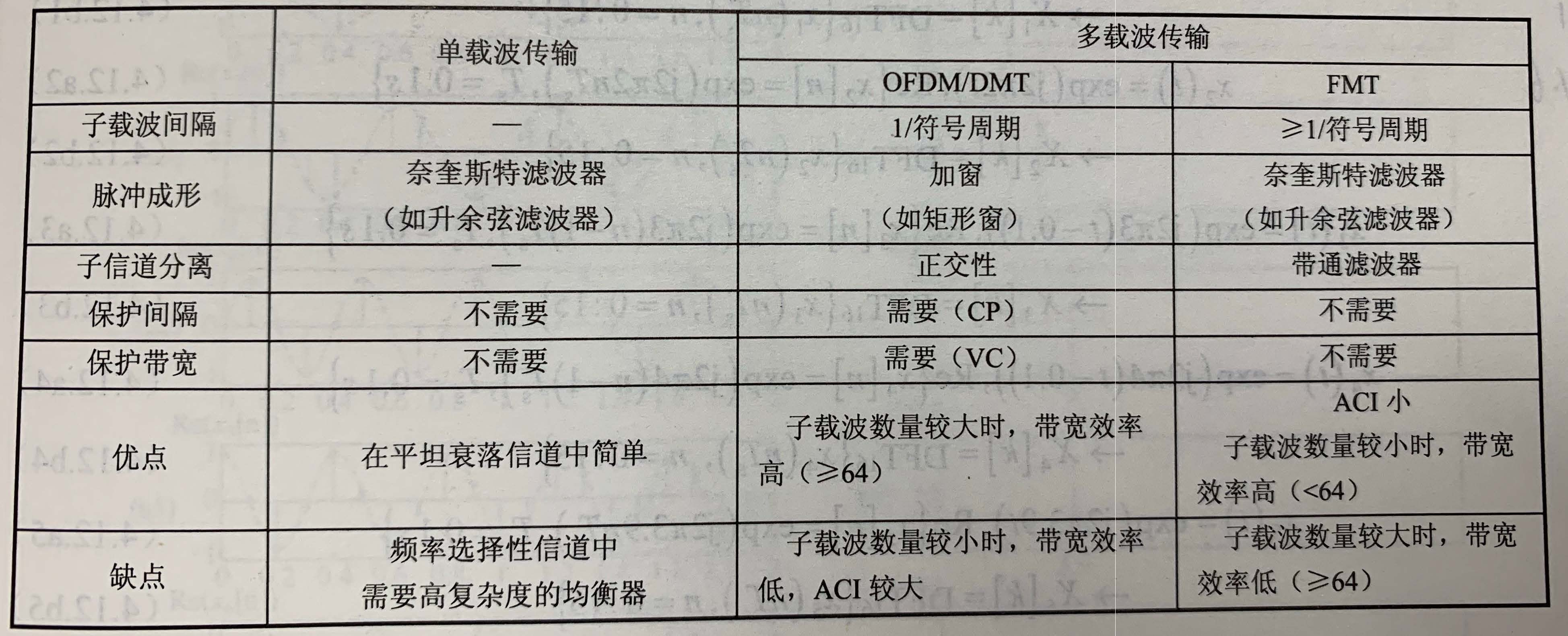FMT - Filtered Multi-tone 用滤波器实现子载波的相互正交(无干扰) ACI - adjacent chanel interference VC - Virtual carrier 作为保护带宽的外侧子载波
ODFM通信链路（发送端）如上图所示, 本身占据整个带宽的短symbols经过串并变换后分散到了各个子载波上. 速度变低也使得他们只占据一个子载波的带宽. 对于第

k

k

路数据 (

k

=

0

,

1

,

.

.

.

,

N

−

1

k=0,1,...,N-1

),我们将symbol乘以载波

e

j

2

π

f

k

t

e^{j2\pi f_k t}

, 其中

f

k

=

k

T

s

f_k=\frac{k}{T_s}

,

0

≤

t

≤

T

s

0 \leq t\leq T_s

. 也就是说, 在复平面上, 第k路信号从复数

s

k

s_k

开始 (

t

=

0

t=0

) 在一个OFDM符号周期

T

s

T_s

内转动

k

k

圈. 经过各个子载波调制后的信号相加得到了OFDM调制信号

∑

k

=

0

N

−

1

s

k

e

j

2

π

f

k

t

\sum_{k=0}^{N-1}s_k e^{j2\pi f_k t}

这是一个连续信号, 因为我们的载波是一个连续的

t

t

. 实际上我们在基带处理时, 载波是用离散的采样点来代表的 (采样时刻

t

=

n

T

s

N

+

τ

,

n

=

0

,

1

,

.

.

.

,

N

−

1

t=n\frac{T_s}{N}+\tau, n=0,1,...,N-1

). 最后得到的信号是一个序列

{

s

n

′

:

n

=

0

,

1

,

.

.

.

,

N

−

1

}

\left\{s'_n:n=0,1,...,N-1\right\}

, 也就是对上面连续信号的采样 (如图中equation所示). 由于发射端我们可以控制，因此载波采样初始相位一般直接可以设置为

τ

=

0

\tau=0

，那么最终得到的信号为

s

n

′

=

1

N

∑

k

=

0

N

−

1

s

k

e

j

2

π

k

n

N

,

n

=

0

,

1

,

2

,

.

.

.

,

N

−

1

s'_n=\frac{1}{N}\sum_{k=0}^{N-1}s_k e^{j2\pi\frac{kn}{N}}, n = 0,1,2,...,N-1

与途中相比，这里我们多加了个

1

/

N

1/N

的归一化过程。从而，这

N

N

个采样点

{

s

n

′

:

n

=

0

,

1

,

.

.

.

,

N

−

1

}

\{s'_n:n=0,1,...,N-1\}

刚刚好是

N

N

个子载波上面放置的

N

N

个symbols

{

s

k

:

k

=

0

,

1

,

.

.

.

,

N

−

1

}

\{s_k:k=0,1,...,N-1\}

的 IDFT. 因此，我们一般认为开始传输的是频域信号，经过“各个子载波调制再加和再sample”相当于做一次IDFT转换成时域信号.  ODFM通信链路（接受端-无信道） 接收端的架构和发送端是对称的，首先是解子载波的调制。这一过程我们利用的是子载波之间的正交性。对离散信号的子载波解调如下（若想写成连续信号形式那就是积分）. 首先，不考虑信道的话，接收信号应该就是发出去的长度为

N

N

的sequence

{

s

n

′

:

n

=

0

,

1

,

.

.

.

,

N

−

1

}

\{s'_n:n=0,1,...,N-1\}

. 那么假设我们解出来的频域sequence是

{

y

m

:

m

=

0

,

1

,

.

.

.

,

N

−

1

}

\{y_m:m=0,1,...,N-1\}

, 则每个symbol的解法如下

y

m

=

∑

n

=

0

N

−

1

s

n

′

e

−

j

2

π

n

m

N

=

∑

n

=

0

N

−

1

(

1

N

∑

k

=

0

N

−

1

s

k

e

j

2

π

k

n

N

)

e

−

j

2

π

n

m

N

=

∑

k

=

0

N

−

1

s

k

1

N

∑

n

=

0

N

−

1

e

j

2

π

(

k

−

m

)

n

N

=

∑

k

=

0

N

−

1

s

k

δ

(

k

−

m

)

=

s

m

y_m=\sum_{n=0}^{N-1}s'_n e^{-j2\pi \frac{nm}{N}} =\sum_{n=0}^{N-1} \left(\frac{1}{N}\sum_{k=0}^{N-1}s_k e^{j2\pi\frac{kn}{N}}\right) e^{-j2\pi \frac{nm}{N}} =\sum_{k=0}^{N-1} s_k \frac{1}{N} \sum_{n=0}^{N-1} e^{j2\pi \frac{(k-m)n}{N}} =\sum_{k=0}^{N-1} s_k \delta(k-m)=s_m

最终得到的

{

y

m

:

m

=

0

,

1

,

.

.

.

,

N

−

1

}

\{y_m:m=0,1,...,N-1\}

是接收序列

{

s

n

′

:

n

=

0

,

1

,

.

.

.

,

N

−

1

}

\{s'_n:n=0,1,...,N-1\}

的 DFT.
注意其实接受序列是由连续信号采样得到，因此真正的采样结果会有一个

τ

\tau

多出来。即，各个子载波与本地载波有一个固定的时偏/相位差，此时

y

m

=

∑

n

=

0

N

−

1

s

n

′

e

−

j

2

π

n

m

N

=

∑

n

=

0

N

−

1

(

1

N

∑

k

=

0

N

−

1

s

k

e

j

2

π

k

n

N

e

j

2

π

k

T

s

τ

)

e

−

j

2

π

n

m

N

=

∑

k

=

0

N

−

1

s

k

e

j

2

π

k

T

s

τ

1

N

∑

n

=

0

N

−

1

e

j

2

π

(

k

−

m

)

n

N

=

∑

k

=

0

N

−

1

s

k

δ

(

k

−

m

)

e

j

2

π

k

T

s

τ

=

s

m

e

j

2

π

m

T

s

τ

y_m=\sum_{n=0}^{N-1}s'_n e^{-j2\pi \frac{nm}{N}} =\sum_{n=0}^{N-1} \left(\frac{1}{N}\sum_{k=0}^{N-1}s_k e^{j2\pi\frac{kn}{N}} e^{j2\pi\frac{k}{T_s}\tau} \right) e^{-j2\pi \frac{nm}{N}}\\ =\sum_{k=0}^{N-1} s_k e^{j2\pi\frac{k}{T_s}\tau} \frac{1}{N} \sum_{n=0}^{N-1} e^{j2\pi \frac{(k-m)n}{N}} =\sum_{k=0}^{N-1} s_k \delta(k-m)e^{j2\pi\frac{k}{T_s}\tau} =s_m e^{j2\pi\frac{m}{T_s}\tau}

此时第

m

m

路解出来的信号除了

s

m

s_m

还会带有一个phase, 这个多余的phase随着

m

m

从 0 到

N

−

1

N-1

刚刚好转一圈.
[ODFM in Multi-path Channel]
信道
我们都知道, 从A点给B点发信息, 信号的强度会随着AB距离的增加而减弱, 这是因为信道的大尺度衰落(包括路径损耗和障碍物引起的阴影衰落). 除了大尺度衰落之外, 还存在小尺度衰落. 它造成的结果是, 1) 即使AB均保持不动, B接受的信号幅度也会不停的波动. 这种现象不仅因为噪声, 还因为现实通信中不可避免的多径效应, 每条路径的时延功率都会发生变化. 2) AB之间存在相对移动时, 即使多径情况不发生变化，多普勒扩展也会使得信号频率发生变化(一个相对速度对应一个多普勒频移), 从而使信道发生变化。
具体来说，信道可用 “相干带宽” 和 “相干时间” 来刻画。 – 相干带宽 相干带宽指的是频域信道保持不变的带宽。显然当信号带宽 < 相干带宽时, 整个信号经历相同的信道 (信道恒定幅度, 线性相位); 当信号带宽 > 相干带宽时, 信号的某些频率分量就会被信道滤波, 从而无法保证一致的发送频谱. 相关带宽的大小显然是看H(f), 也就是

h

(

τ

)

h(\tau)

的傅里叶变换. 最终最大多径时延和相干带宽成反比. 传输的符号不管长短都会受到多径的影响,只要有多径就会有ISI, 但是只要符号时长

T

s

T_s

大于最大多径时延, 信号带宽就会小于相干带宽, 信号就不会经历频率选择性衰落信道 (从时域分析,我不理解为什么符号时长

T

s

T_s

大于最大多径时延就没有就没有频率选择性衰落,比如只有两径, 我感觉第二径不管时延多大对原信号的decode影响都是一样的,除非没有时延相干叠加. 但是书上会说只要

T

s

T_s

大于最大多径时延,当前符号就不会对下个符号造成太大影响, ISI不显著).
– 相干时间 对于静止的物体来说, 相干带宽已经可以刻画信道的特性, 但是通常用户和BS之间会有相对移动, 而一个相对移动速度对应着一个多普勒频偏. 多普勒频偏会使得载波发生偏移从而变相的让发送信号的带宽变大, 而变大的幅度取决于最大相对速度. 显然, 发送端在移动时, 根据速度的变化接收端收到的频率也不同很容易超过相干带宽导致信道发生变化. 最大速度决定了最大多普勒频移, 也就决定了相干时间大小. 运动得越快, 相干时间越小 (信道越容易发生变化). 因此符号时长太长了更容易经历大于相干时间从而经历时间选择性衰落信道.
CP for ICI and ISI
OFDM 中, 子载波是在一个长OFDM符号上连续变化的 (即

T

s

T_s

内只会调制一个符号), 因此符号周期应该看一个OFDM符号的周期

T

s

T_s

. OFDM系统中需要避免的两种干扰是 ICI (子载波间干扰) 和 ISI (OFDM符号间干扰). 造成ICI的原因是子载波间的正交性无法得到满足, 而ISI主要是因为多径造成前后symbol相互混叠.
为了对抗多径, OFDM符号一般会附加一段循环前缀CP. CP的作用是, 即使有多径, 只要小于CP长度, 我们还是能成功采样出完整的一个OFDM符号从而消除ICI. 但是实际系统中由于存在定时偏差STO, 最终选取FFT框时可能会选在任意位置. 假设CP长度为

T

c

T_c

, ODFM符号长度为

T

s

T_s

, 最大多径时延是

T

τ

T_\tau

. 那么– 如果FFT框起始点在

[

0

,

T

τ

]

[0,T_\tau]

, 则我们会得到一个完整的OFDM符号, 因此不会有ICI; 但是会有ISI因为上一符号会有干扰. – 如果FFT框起始点在

[

T

τ

,

T

c

]

[T_\tau,T_c]

, 则我们会得到一个完整的OFDM符号, 因此不会有ICI; 上一符号也没干扰因此也没有ISI. – 如果FFT框起始点在

[

T

c

,

T

s

]

[T_c,T_s]

, 则我们连一个完整的OFDM符号都得不到, 因此有ICI; 而且也有ISI因为下一符号会有干扰.
Independent channels for Sub-carriers
在上一节中, 我们已经由预发送的频域符号

s

k

s_k

得到发送端的基带传输信号

s

n

′

s'_n

. 为了书写更明确, 我们令

x

[

n

]

=

s

n

′

x[n] = s'_n

,

S

[

k

]

=

s

k

S[k]=s_k

, 则

x

[

n

]

=

1

N

∑

k

=

0

N

−

1

S

[

k

]

e

j

2

π

k

n

N

,

n

=

0

,

1

,

2

,

.

.

.

,

N

−

1

x[n]=\frac{1}{N}\sum_{k=0}^{N-1}S[k] e^{j2\pi\frac{kn}{N}}, n = 0,1,2,...,N-1

其中,

n

n

是对模拟信号的采样index, 每个采样点都是

N

−

1

N-1

个载波的叠加.
考虑基带离散信道

{

h

[

n

]

,

n

=

0

,

1

,

.

.

.

,

∞

}

\{h[n], n = 0, 1,...,\infty\}

, 接收信号为

y

[

n

]

=

h

[

n

]

∗

x

[

n

]

+

w

[

n

]

=

∑

m

=

0

∞

h

[

m

]

x

[

n

−

m

]

+

w

[

n

]

=

∑

m

=

0

∞

h

[

m

]

1

N

∑

k

=

0

N

−

1

S

[

k

]

e

j

2

π

k

(

n

−

m

)

N

+

w

[

n

]

y[n]=h[n]\ast x[n] + w[n]=\sum_{m=0}^{\infty} h[m]x[n-m] +w[n] \\ =\sum_{m=0}^{\infty} h[m]\frac{1}{N}\sum_{k=0}^{N-1}S[k] e^{j2\pi\frac{k(n-m)}{N}} +w[n]

对

y

[

n

]

y[n]

这个N点序列进行离散傅里叶变换, 得到

Y

[

k

′

]

=

∑

n

=

0

N

−

1

y

[

n

]

e

−

j

2

π

n

k

′

N

=

∑

n

=

0

N

−

1

(

∑

m

=

0

∞

h

[

m

]

1

N

∑

k

=

0

N

−

1

S

[

k

]

e

j

2

π

k

(

n

−

m

)

N

)

e

−

j

2

π

n

k

′

N

+

W

[

k

′

]

=

∑

n

=

0

N

−

1

(

∑

m

=

0

∞

h

[

m

]

e

j

2

π

−

k

m

N

)

1

N

∑

k

=

0

N

−

1

S

[

k

]

e

j

2

π

(

k

−

k

′

)

n

N

+

W

[

k

′

]

=

1

N

∑

n

=

0

N

−

1

∑

k

=

0

N

−

1

H

[

k

]

S

[

k

]

e

j

2

π

(

k

−

k

′

)

n

N

+

W

[

k

′

]

Y[k']=\sum_{n=0}^{N-1}y[n]e^{-j2\pi\frac{nk'}{N}} =\sum_{n=0}^{N-1} \left(\sum_{m=0}^{\infty} h[m]\frac{1}{N}\sum_{k=0}^{N-1}S[k] e^{j2\pi\frac{k(n-m)}{N}}\right)e^{-j2\pi\frac{nk'}{N}} +W[k'] \\ =\sum_{n=0}^{N-1} \left(\sum_{m=0}^{\infty} h[m] e^{j2\pi\frac{-km}{N}}\right) \frac{1}{N}\sum_{k=0}^{N-1}S[k] e^{j2\pi\frac{(k-k')n}{N}} + W[k'] =\frac{1}{N}\sum_{n=0}^{N-1} \sum_{k=0}^{N-1}H[k] S[k] e^{j2\pi\frac{(k-k')n}{N}} +W[k']

变换成DFT套IDFT的形式解

=

∑

n

=

0

N

−

1

(

1

N

∑

k

=

0

N

−

1

H

[

k

]

S

[

k

]

e

j

2

π

k

n

N

)

e

j

2

π

−

k

′

n

N

+

W

[

k

′

]

=

H

[

k

′

]

S

[

k

′

]

+

W

[

k

′

]

=\sum_{n=0}^{N-1} \left(\frac{1}{N}\sum_{k=0}^{N-1}H[k] S[k] e^{j2\pi\frac{kn}{N}} \right) e^{j2\pi\frac{-k'n}{N}} +W[k'] =H[k']S[k']+W[k']

.
用子载波正交性解

=

∑

k

=

0

N

−

1

H

[

k

]

S

[

k

]

1

N

∑

n

=

0

N

−

1

e

j

2

π

(

k

−

k

′

)

n

N

+

W

[

k

′

]

=

∑

k

=

0

N

−

1

H

[

k

]

S

[

k

]

δ

(

k

−

k

′

)

+

W

[

k

′

]

=

H

[

k

′

]

S

[

k

′

]

+

W

[

k

′

]

= \sum_{k=0}^{N-1}H[k] S[k] \frac{1}{N}\sum_{n=0}^{N-1}e^{j2\pi\frac{(k-k')n}{N}} +W[k'] = \sum_{k=0}^{N-1}H[k] S[k] \delta(k-k')+W[k']=H[k']S[k']+W[k']

结论: 从发送端IDFT前在子载波上放符号，到接受端DFT之后解出的频域信号，中间 “IDFT+多径信道+DFT” 可以看作 各子载波单独过信道，用一个抽头为1的均衡器即可以去除信道

{

h

(

n

)

:

n

=

0

,

1

,

.

.

.

,

∞

}

\{h(n): n=0,1,...,\infty\}

的影响。 多径信道对信号的作用是卷积，在上CP之后多径信道的作用就变成循环卷积了。对于一个序列来说，时域循环卷积等于频域（IDFT）对应点乘
With STO
在以上的推导中，其实我们是假设接收端的perfect sampling的。我们发送的信号是对载波调制信号在

{

n

T

s

/

N

:

n

=

0

,

1

,

.

.

.

,

∞

}

\{nT_s/N:n=0,1,...,\infty\}

处的采样结果，接收信号也直接用这个发送信号与信道卷积得到
子载波调制

→

\rightarrow

发送端采样

→

\rightarrow

信道卷积

→

\rightarrow

接收信号

→

\rightarrow

FFT
但是实际情况中，由于STO我们接受端采样不可能那么精准，因此更general的采样时刻应该是

{

(

n

−

n

′

)

T

s

N

+

τ

:

n

=

0

,

1

,

.

.

.

,

N

−

1

}

\{\frac{(n-n')T_s}{N}+\tau:n=0,1,...,N-1\}

, 其中

n

′

n'

是整数倍采样偏差，

τ

\tau

是小数倍采样偏差

0

≤

τ

≤

T

s

N

0\leq\tau\leq \frac{T_s}{N}

. 而且注意， 这里

n

=

0

,

1

,

.

.

.

,

N

−

1

n=0,~1,...,~N-1

, 则有

n

−

n

′

=

−

n

′

,

1

−

n

′

,

2

−

n

′

,

.

.

.

,

−

1

,

0

,

1

,

.

.

.

,

N

−

1

−

n

′

n-n'=-n',~1-n',~2-n', ~..., ~-1, ~0, ~1, ~...,~N-1-n'

。 由于有CP的存在，我们进一步有

n

−

n

′

=

N

−

n

′

,

N

−

n

′

+

1

,

.

.

.

,

N

−

1

,

0

,

1

,

2

,

.

.

.

,

N

−

1

−

n

′

n-n'=N-n',~N-n'+1,...,~N-1,~0,~1,~2,~...,~N-1-n'

.
因为信道卷积只是几个径的叠加，因此我们把这个采样的不精准放在发送端，即让发送端采样就已经不精准了，此时

x

[

n

]

=

1

N

∑

k

=

0

N

−

1

S

[

k

]

e

j

2

π

k

(

n

−

n

′

)

N

e

j

2

π

k

T

s

τ

,

n

=

0

,

1

,

2

,

.

.

.

,

N

−

1

x[n]=\frac{1}{N}\sum_{k=0}^{N-1}S[k] e^{j2\pi\frac{k(n-n')}{N}} e^{j2\pi\frac{k}{T_s}\tau}, n = 0,1,2,...,N-1

注意，采样不精准仅仅该变每一路

S

[

k

]

S[k]

乘的相位而已。其实对一个OFDM的符号采样，每一个采样点唯一的不同就是每一路

S

[

k

]

S[k]

乘的载波相位不同（随着时间一直旋转）。
接收信号是信道卷积而来：

y

[

n

]

=

h

[

n

]

∗

x

[

n

]

+

w

[

n

]

=

∑

m

=

0

∞

h

[

m

]

x

[

n

−

m

]

+

w

[

n

]

=

∑

m

=

0

∞

h

[

m

]

1

N

∑

k

=

0

N

−

1

S

[

k

]

e

j

2

π

k

(

n

−

n

′

−

m

)

N

e

j

2

π

k

T

s

τ

+

w

[

n

]

,

n

=

0

,

1

,

2

,

.

.

.

,

N

−

1

y[n]=h[n]\ast x[n] + w[n]=\sum_{m=0}^{\infty} h[m]x[n-m] +w[n] \\ =\sum_{m=0}^{\infty} h[m]\frac{1}{N}\sum_{k=0}^{N-1}S[k] e^{j2\pi\frac{k(n-n'-m)}{N}}e^{j2\pi\frac{k}{T_s}\tau} +w[n],~~~~n = 0,1,2,...,N-1

对

y

[

n

]

y[n]

这个N点序列进行离散傅里叶变换, 得到

Y

[

k

′

]

=

∑

n

=

0

N

−

1

y

[

n

]

e

−

j

2

π

n

k

′

N

=

∑

n

=

0

N

−

1

(

∑

m

=

0

∞

h

[

m

]

1

N

∑

k

=

0

N

−

1

S

[

k

]

e

j

2

π

k

(

n

−

n

′

−

m

)

N

e

j

2

π

k

T

s

τ

)

e

−

j

2

π

n

k

′

N

+

W

[

k

′

]

=

∑

n

=

0

N

−

1

(

∑

m

=

0

∞

h

[

m

]

e

j

2

π

−

k

m

N

)

1

N

∑

k

=

0

N

−

1

S

[

k

]

e

j

2

π

(

k

−

k

′

)

n

N

e

−

j

2

π

k

n

′

N

e

j

2

π

k

T

s

τ

+

W

[

k

′

]

=

1

N

∑

n

=

0

N

−

1

∑

k

=

0

N

−

1

H

[

k

]

S

[

k

]

e

j

2

π

(

k

−

k

′

)

n

N

e

−

j

2

π

k

n

′

N

e

j

2

π

k

T

s

τ

+

W

[

k

′

]

=

∑

k

=

0

N

−

1

H

[

k

]

S

[

k

]

δ

(

k

−

k

′

)

e

−

j

2

π

k

n

′

N

e

j

2

π

k

T

s

τ

+

W

[

k

′

]

=

H

[

k

′

]

e

−

j

2

π

k

′

n

′

N

e

j

2

π

k

′

T

s

τ

S

[

k

′

]

+

W

[

k

′

]

Y[k']=\sum_{n=0}^{N-1}y[n]e^{-j2\pi\frac{nk'}{N}} =\sum_{n=0}^{N-1} \left( \sum_{m=0}^{\infty} h[m]\frac{1}{N}\sum_{k=0}^{N-1}S[k] e^{j2\pi\frac{k(n-n'-m)}{N}}e^{j2\pi\frac{k}{T_s}\tau} \right)e^{-j2\pi\frac{nk'}{N}} +W[k'] \\ =\sum_{n=0}^{N-1} \left(\sum_{m=0}^{\infty} h[m] e^{j2\pi\frac{-km}{N}}\right) \frac{1}{N}\sum_{k=0}^{N-1}S[k] e^{j2\pi\frac{(k-k')n}{N}}e^{-j2\pi\frac{kn'}{N}}e^{j2\pi\frac{k}{T_s}\tau} + W[k'] \\ =\frac{1}{N}\sum_{n=0}^{N-1} \sum_{k=0}^{N-1}H[k] S[k] e^{j2\pi\frac{(k-k')n}{N}}e^{-j2\pi\frac{kn'}{N}}e^{j2\pi\frac{k}{T_s}\tau} +W[k'] \\ = \sum_{k=0}^{N-1}H[k] S[k] \delta(k-k') e^{-j2\pi\frac{kn'}{N}}e^{j2\pi\frac{k}{T_s}\tau} +W[k']\\ =H[k'] e^{-j2\pi\frac{k'n'}{N}}e^{j2\pi\frac{k'}{T_s}\tau} S[k'] +W[k']

. 结论: “IDFT+多径信道+DFT+无ISI和ICI的采样偏差” 仍可以看作子载波单独过信道，区别是频域信道在本身多径信道的基础上多了一个相位
与无循环移位的比较
当然，我们也可以把

x

[

n

]

x[n]

直接定义成

x

[

n

]

=

1

N

∑

k

=

0

N

−

1

S

[

k

]

e

j

2

π

k

n

N

e

j

2

π

k

T

s

τ

,

n

=

N

−

n

′

,

.

.

.

,

N

−

1

,

0

,

1

,

2

,

.

.

.

,

N

−

1

−

n

′

.

x[n]=\frac{1}{N}\sum_{k=0}^{N-1}S[k] e^{j2\pi\frac{kn}{N}} e^{j2\pi\frac{k}{T_s}\tau}, n = N-n', ...,N-1,0,1,2,...,N-1-n'.

实际上就相当于对

x

[

n

]

x[n]

做了一次循环移位，比如由

{

x

[

0

]

,

x

[

1

]

,

x

[

2

]

,

x

[

3

]

}

\{x, x, x, x\}

变成了

{

x

[

3

]

,

x

[

0

]

,

x

[

1

]

,

x

[

2

]

}

\{x, x, x, x\}

(

n

′

=

1

n'=1

). 第一种写法还是延续之前的index

n

n

, 而第二种重新定义了新的index

n

n

. 我们采用第一种写法。则有
展开全文• ## 直观图解OFDM原理

万次阅读 多人点赞 2018-09-11 11:17:52
人生如幻，不知怎么的碰（bei）巧（ju）和OFDM对上了。 这是一个大坑，很大又绕不过去的坑，不是三言两语能够解释清楚的，目测需要融合贯通理解数学的三角函数，傅里叶，通原的检波，调制，信道特征，信号处理的...
介绍
人生如幻，不知怎么的碰（bei）巧（ju）和OFDM对上了。  这是一个大坑，很大又绕不过去的坑，不是三言两语能够解释清楚的，目测需要融合贯通理解数学的三角函数，傅里叶，通原的检波，调制，信道特征，信号处理的采样，电子元件特征等等知识才能有个大概了解。详细可以参考专（da）业（bu）书（tou）：L. Hanzo et al., OFDM and MC-CDMA for Broadband Multi-User Communications, Wiley, 2003.
时间精力有限，先来个直观图解，也好方便以后深入。  下文主要参考另一篇很好的入门文章作3D图：给”小白”图示讲解OFDM的原理 - CSDN博客
正交检波 Orthogonality
不同的正弦倍谐波是正交的。意思是不同频率倍数的正弦波积分之后为零。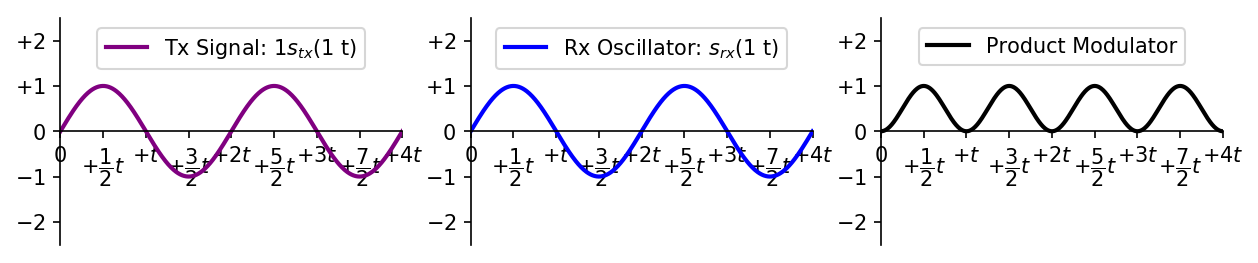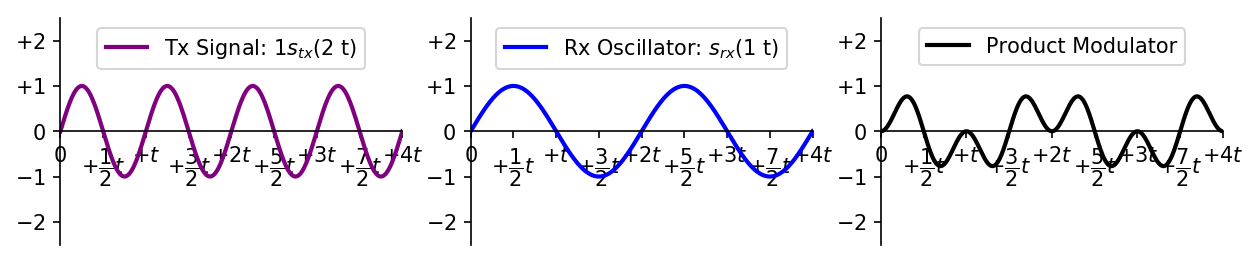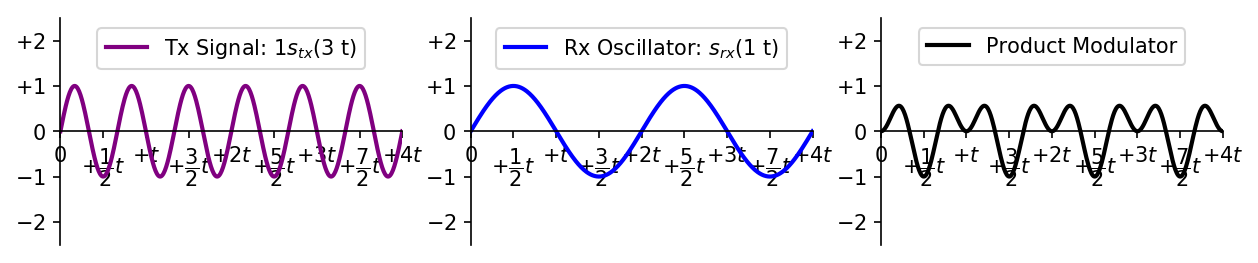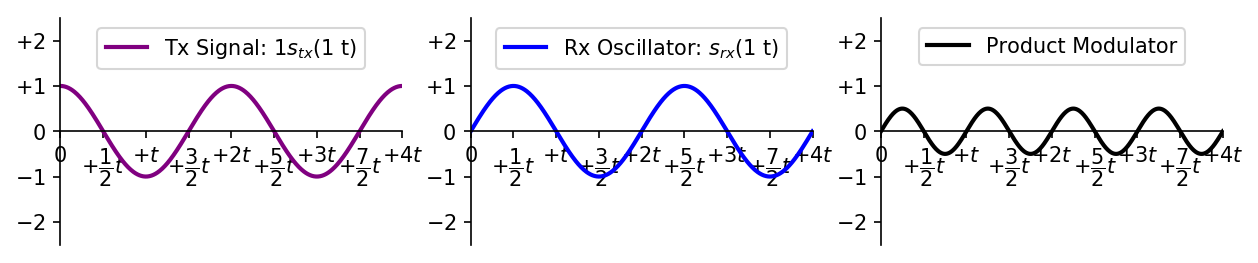如前面三图所示只有频率相同的时候，积分器的结果不为零。频率不同的时候积分在x轴上下面积相同，积分结果是零。  另外，sin和cos（也就是90度相位差）的两个信号的积分结果一样是零，所以sin和cos也是正交的。
谐波的频分复用 Frequency Division Multiplexing
了解不同频率的正弦余弦的正交性这一点之后，我们就可以同时利用这个特征同时发送不同频率的正弦余弦波。而在接收器一侧，使用不同频率积分器积分之后，我们是有能力分辨出不同频率谐波。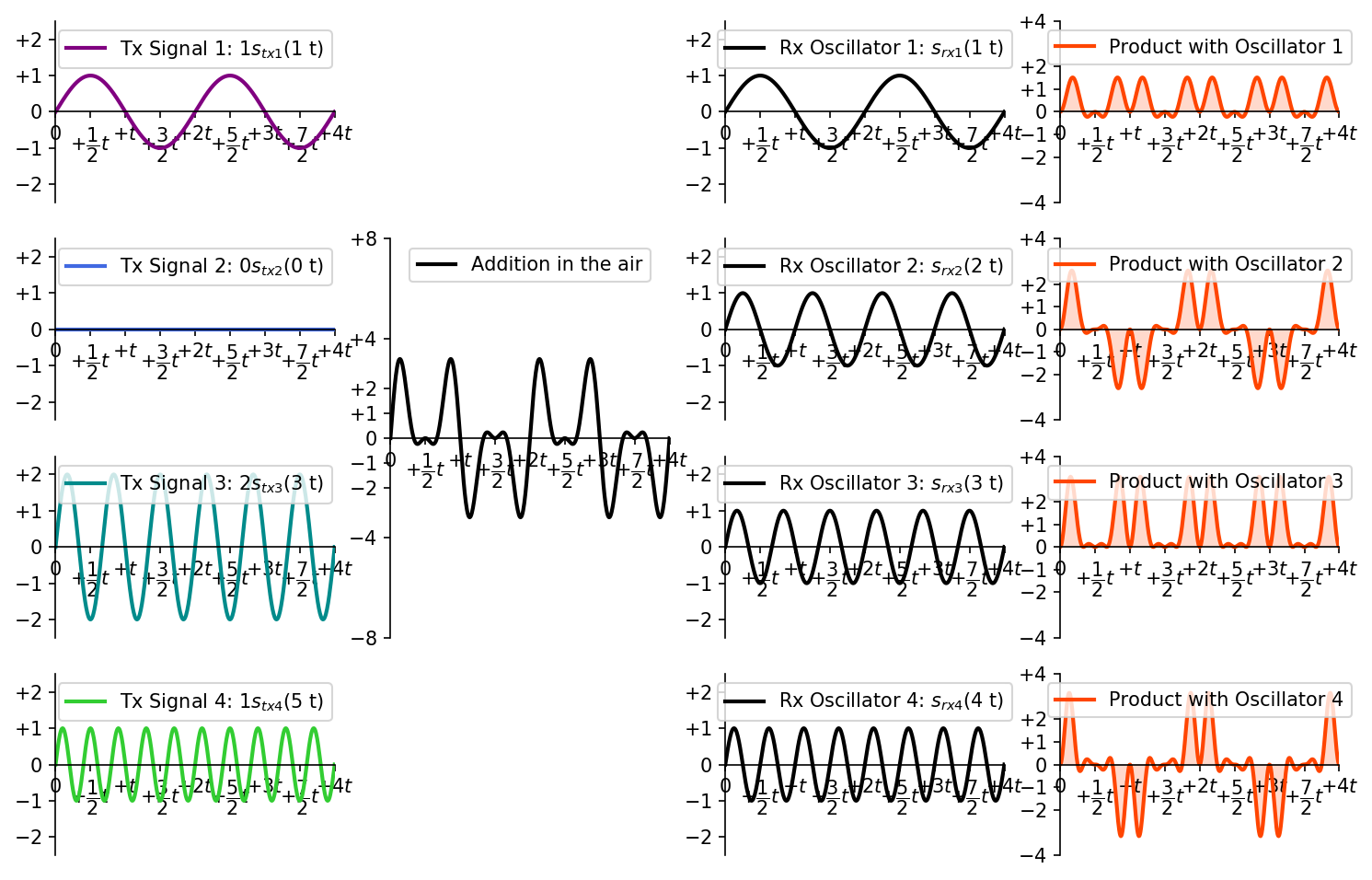上图中，1sin1t+0sin2t+2sin3t+0sin4t+1sin5t的信号（10201）同时发送。接收器里面，sin2t和sin4t的积分器输出为零，而sin1t和sin3t的积分器输出不为零，而且sin3t的信号功率大。接收器检测到了（1020），sin5t的信号1检测不到。
时域和频域
频域和时域其实画成3D就很好理解了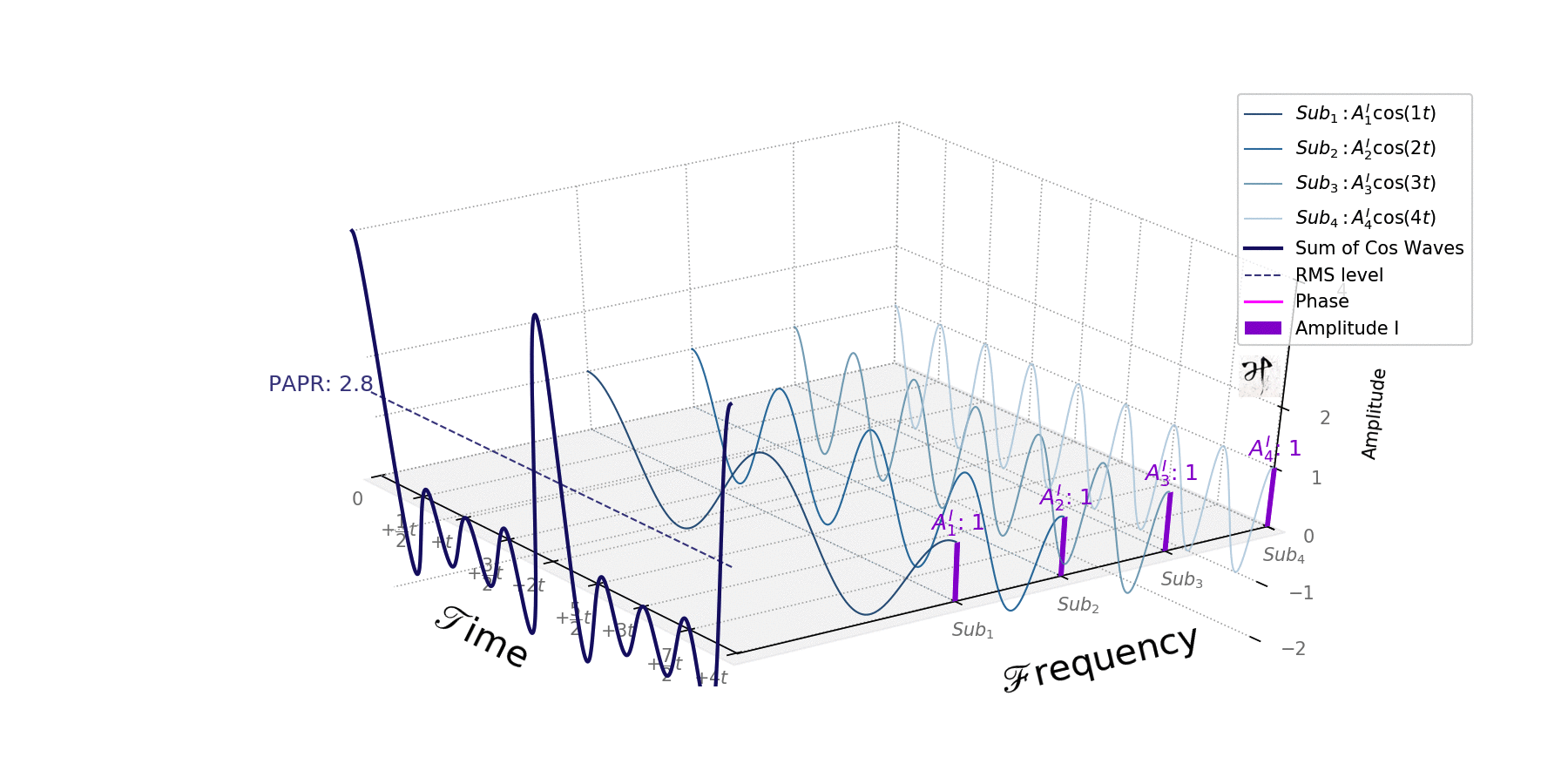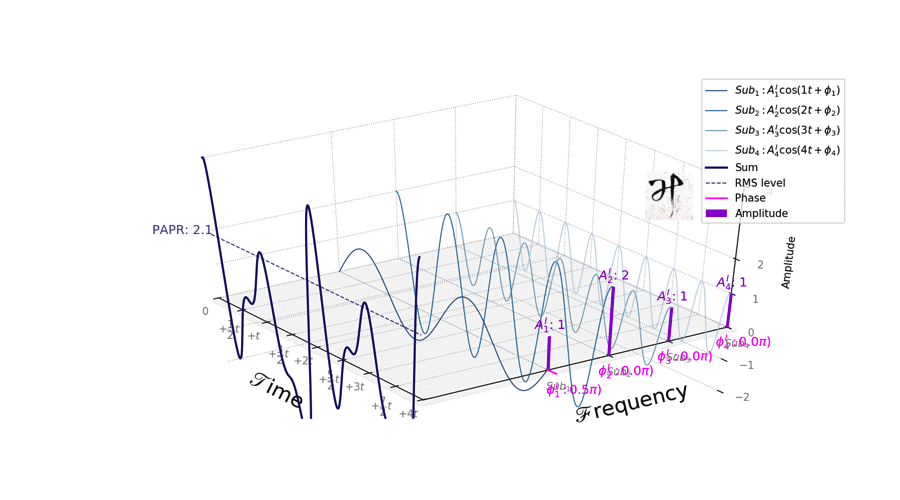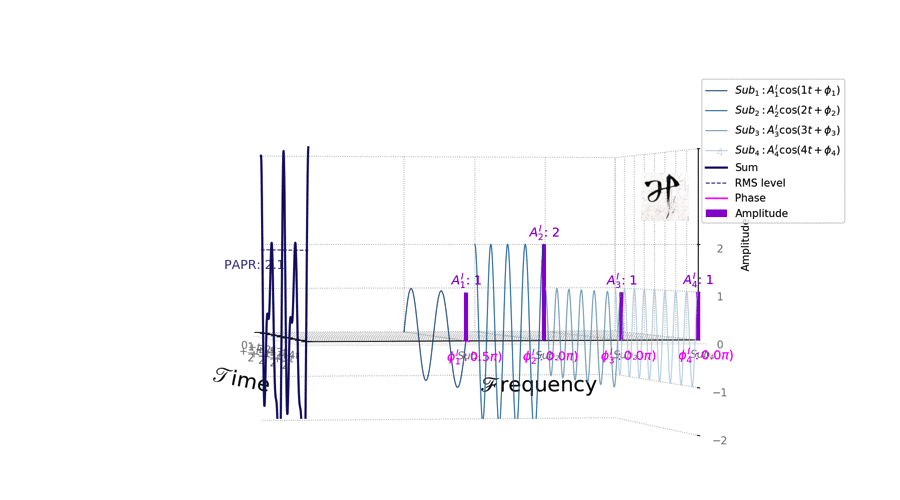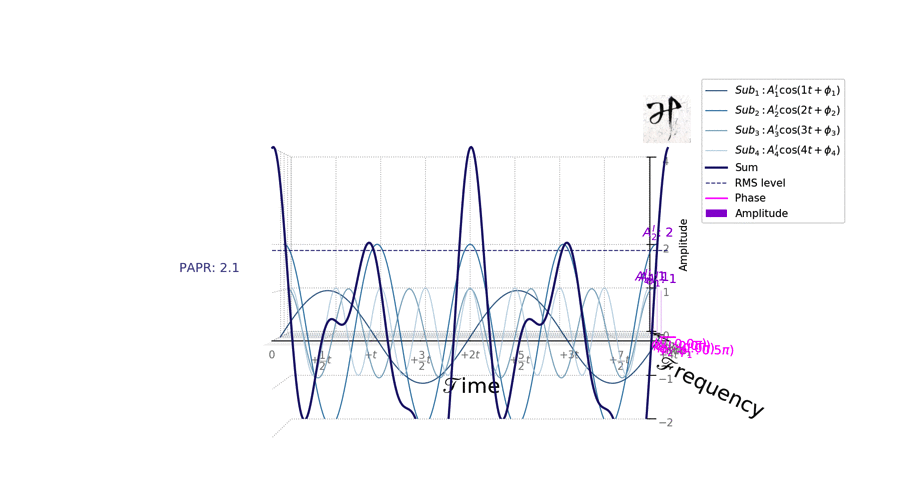除了振幅，也可以改变相位用来传输数据。这个时候相当于APSK吧。  换个角度看频域和时域是不是清楚了？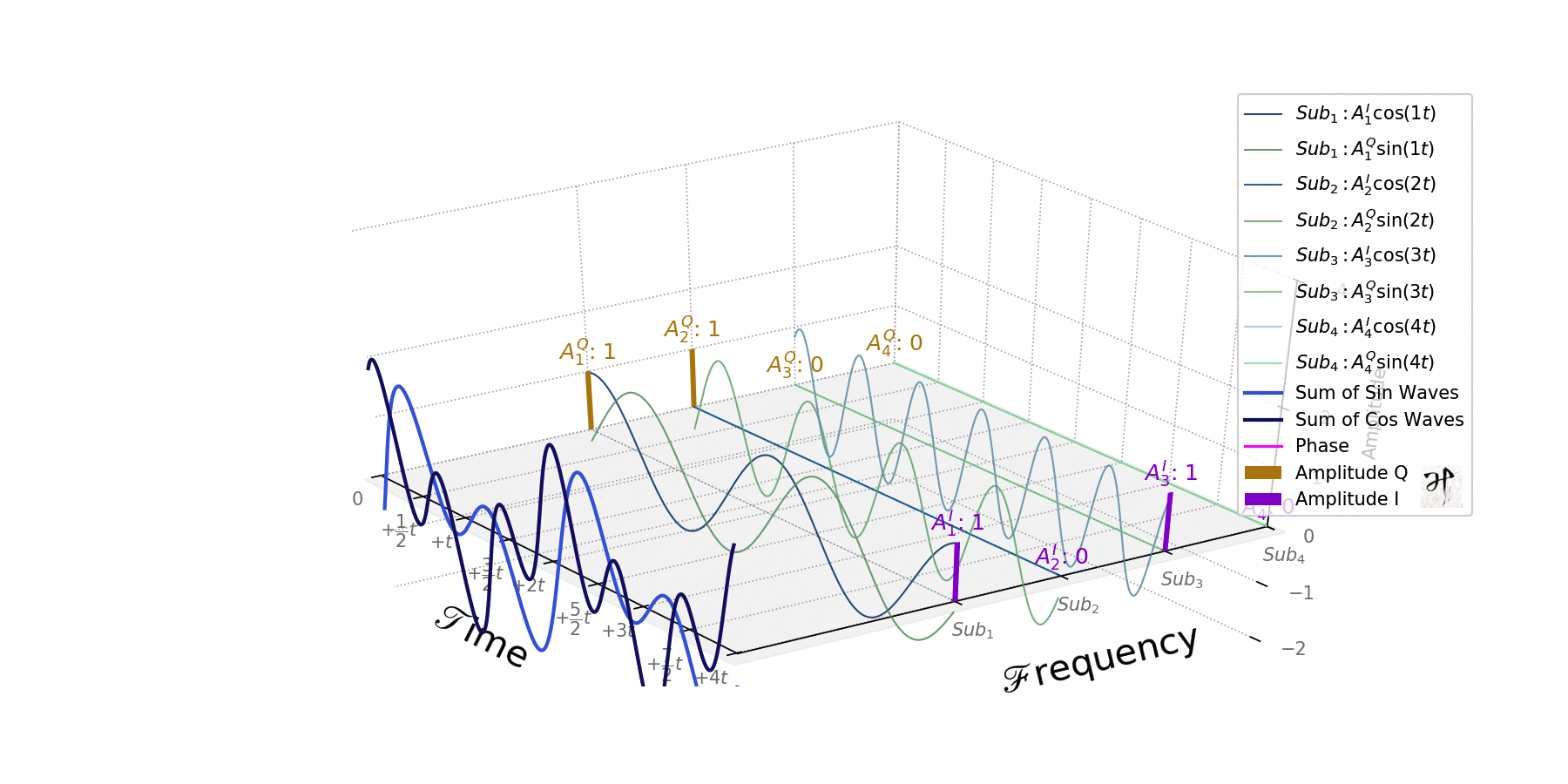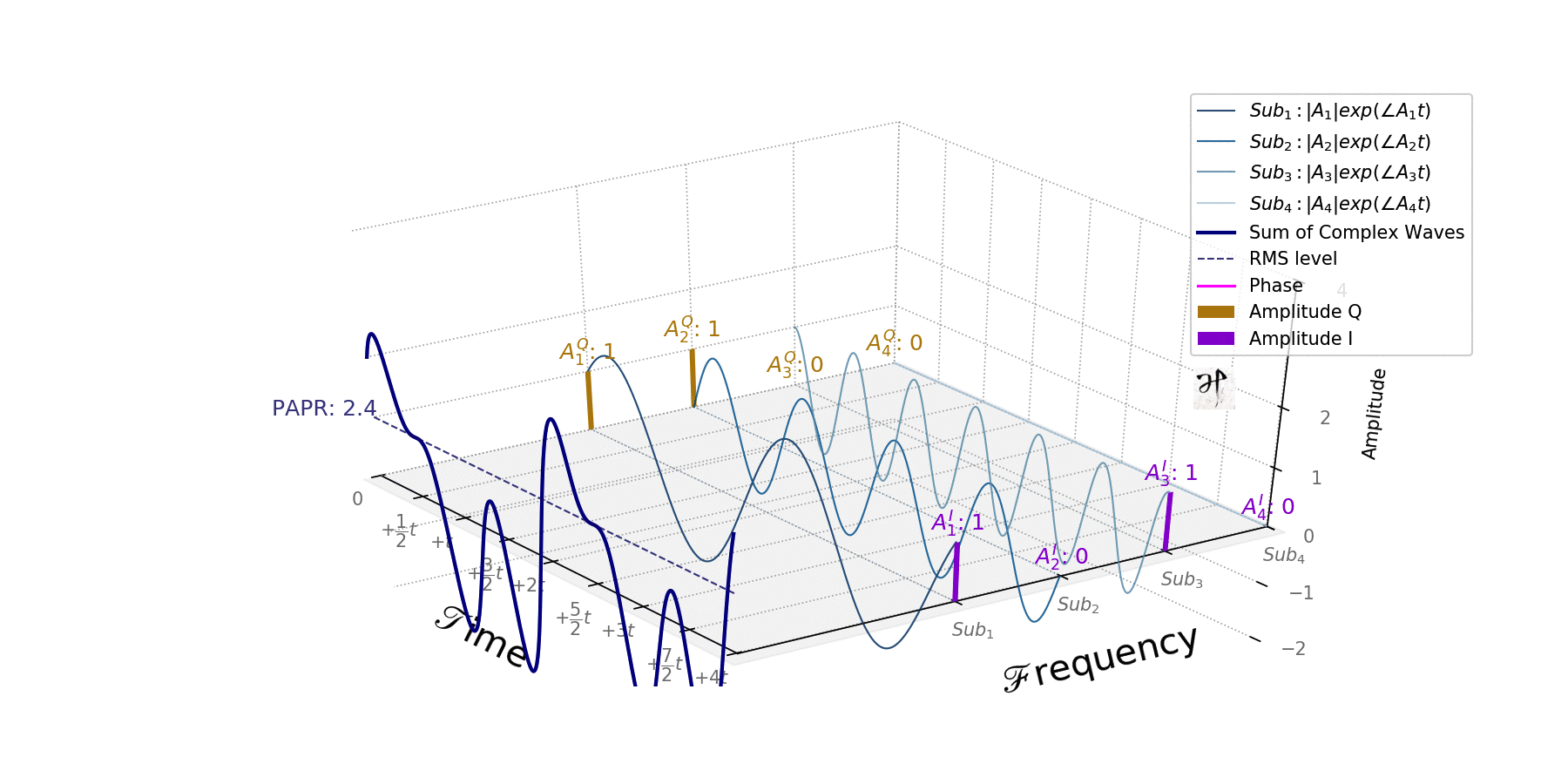别忘了，其实我们可以用一个复数信号来代替同频率的一个正弦波和一个余弦波的和。也就是欧拉公式丢掉右边部分的i。通信老师会给你说这都是为了方便，用一个复数代替两个三角函数就可以将很多运算公式简约很多了。  传递的信息变成了（A1,A2,A3,A4），这里面的每一个symbol的Ak都是复数。
然后仔细一看在时域的复信号的求和公式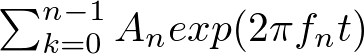这不就是一个傅里叶级数么！（该复习的复习去）  然后傅里叶级数不是可以用IDFT/IFFT来计算么！
由此我们就可以用对复数symbol的数列进行IDFT直接得到时域的发送信号了。
系统架构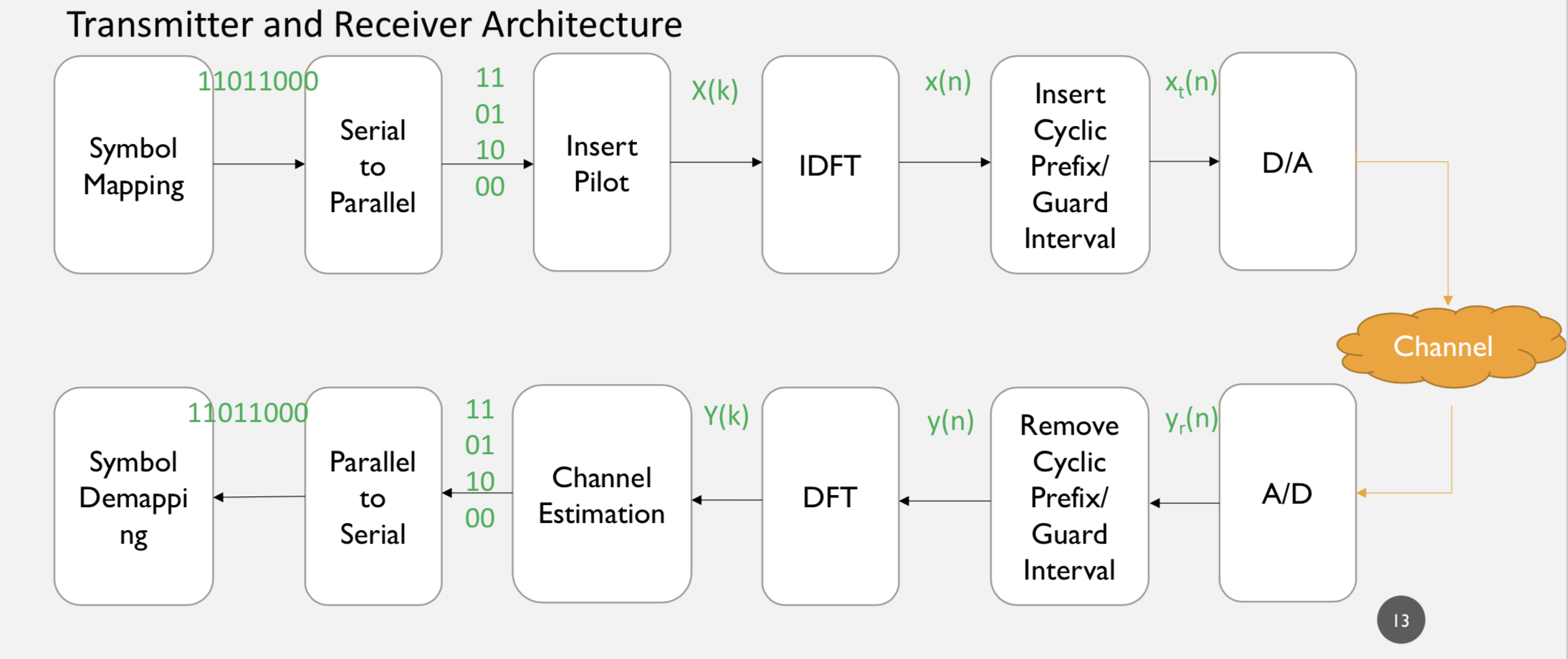动图都了解之后，系统架构就很直白了。  以传输11011000为例，首先分成11,01,10,00并列的四个symbol，然后加入pilot（以后有空再谈），对四个复数symbol进行IDFT_IFFT就可以得到时域的实际发送的信号，然后再加入CP_GI，最后转换成数字数据，升到中频/高频后发送。接收器是倒过来的操作。
采样方面来看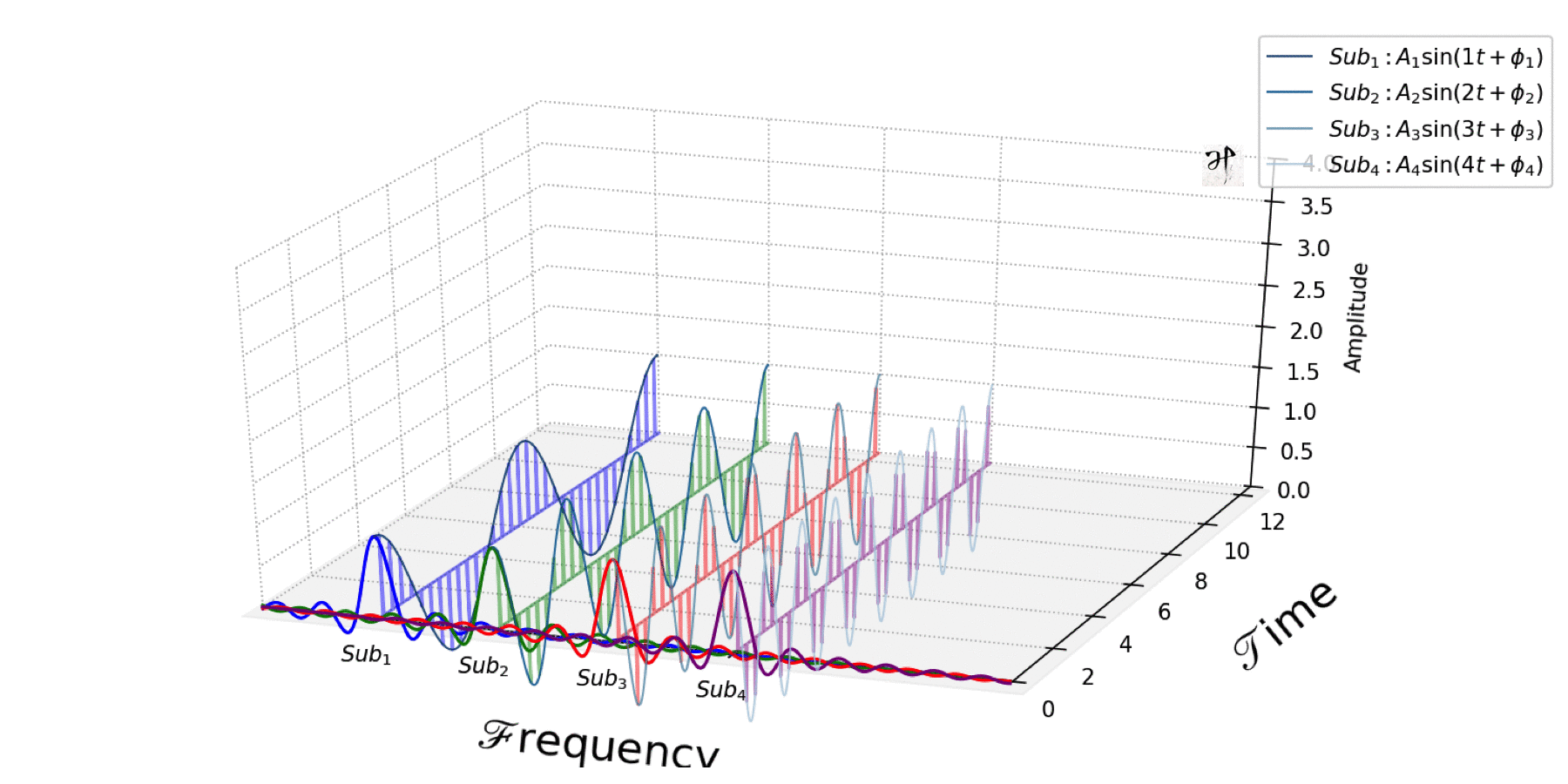采样方面，采样间隔决定了频域上面sinc函数的零点之间间隔。
看上图第一个和第四个子载波，改变采样频率的话，第一个子载波的频域sinc函数会和其他子载波重叠，改变子载波频率距离的话，第四个子载波的采样数据也会和其他子载波重叠。
所以，采样间隔和子载波频率间隔两者必须要符合一定规则。这样采样后的频域数据sinc函数的零点会刚好和其他子载波的，频率以及零点重合，也就是说只有这样子载波之间才能不影响，保持正交。
其他特征
PAPR
这个看上面的gif就可以很好理解了。  PAPR是合成后的发送信号的峰值和平均值的比。  传输信息的时候，每个子载波的频幅和相位可以假设为独立随机事件。所有子载波频幅和相位出现一致的情况下（所有子载波的symbol一样），叠加而成的合成波会出现非常大的峰值，可以超出平均值很多dB，总体来说子载波越多，PAPR越大。  而电子元件的放大器的线性响应范围是有限的，也就是说我们的symbol组合要求一个超出线性范围的峰值的时候，放大器实际上是做不到的。
GI & CP
有空待续
CFO & SFO
动图代码
https://github.com/hanspond/visual_comm
可以手动调整信号，直观观察OFDM信号变化参考
给”小白”图示讲解OFDM的原理 - CSDN博客
正交频分复用（OFDM）原理
L. Hanzo et al., OFDM and MC-CDMA for Broadband Multi-User Communications, Wiley, 2003.
Weinstein, S. B. (2009). The history of orthogonal frequency-division multiplexing. IEEE Communications Magazine, 47(11), 26–35. https: doi. org_10. 1109_MCOM. 2009. 5307460
վ HᴗP ի
展开全文可视化 python
• 文章目录（一）OFDM的概念（二）OFDM的基本原理2.1 概念说明2.2 OFDM的 （一）OFDM的概念   OFDM，英语全称为Orthogonal Frequency Division Multiplexing，中文全称为正交频分复用技术，实际上是MCM(Multi ...


文章目录
（一）OFDM的概念（二）OFDM的基本原理2.1 概念说明2.2 OFDM原理及实现OFDM的基带信号表达式：OFDM的调制框图：OFDM调制与IDFT：

（一）OFDM的概念
OFDM，英语全称为Orthogonal Frequency Division Multiplexing，中文全称为正交频分复用技术，实际上是MCM(Multi Carrier Modulation)，多载波调制的一种。   OFDM通过频分复用实现高速串行数据的并行传输。它的调制和解调分别是基于IFFT和FFT来实现的。
（二）OFDM的基本原理

OFDM的主要思想是：将信道分成若干正交子信道，将高速数据信号转换成并行的低速子数据流，调制到在每个子信道上进行传输。正交信号可以通过在接收端采用相关技术来分开，这样可以减少子信道之间的相互干扰。每个子信道上的信号带块小于信道的相关带宽，因此每个子信道上可以看成平坦性衰落，从而可以消除码间串扰，而且由于每个子信道的带宽仅仅是原信道带宽的一小部分，信道均衡变得相对容易。

2.1 概念说明
平坦性衰落：   多路信号到达接收机的时间有先有后，即有相对时间延迟。如果这些相对时延远小于一个符号的时间，则可以认为多路信号几乎是同时到达接收机的，这种情况下多径不会造成符号间的干扰。这种衰落称为平坦衰落，因为这种信道的频率响应响应在所用的频段内是平坦的。 多径效应:   同一信号的不同分量到达的时间不同 时延扩展：   多径效应引起的接受信号脉冲宽度扩展的现象称为时延扩展。时延扩展（多径信号最快和最慢的时间差）小于码元周期可以避免码间串扰，超过一个码元周期需要用分集接受，均衡算法来接受。 相关带宽：   相关带宽内各频率分量的衰落是一致的也叫相关的，不会失真。载波宽度大于相关带宽就会引起频率选择性衰落使接收信号失真。
2.2 OFDM原理及实现

详见宝藏博主“king阿金”的博客   正交频分复用(OFDM)原理及实现

以下是本人记忆重点。   使

s

i

n

(

t

)

sin(t)

的幅度为

a

a

，

s

i

n

(

2

t

)

sin(2t)

的幅度为

b

b

，也就是将

a

a

调制于

s

i

n

(

t

)

sin(t)

，将

b

b

调制于

s

i

n

(

2

t

)

sin(2t)

，同时传输这两个调制了信号的正弦波（子载波）：

a

×

s

i

n

(

t

)

+

b

×

s

i

n

(

2

t

)

a\times sin(t)+b\times sin(2t)

，在接收时又分别对两路子载波进行积分，也就是：

∫

0

2

π

(

a

s

i

n

(

t

)

+

b

s

i

n

(

2

t

)

)

×

s

i

n

(

t

)

d

t

=

a

∫

0

2

π

s

i

n

2

(

t

)

d

t

\int_0^{2\pi}(asin(t)+bsin(2t))\times sin(t)dt=a\int_0^{2\pi}sin^2(t)dt

∫

0

2

π

(

a

s

i

n

(

t

)

+

b

s

i

n

(

2

t

)

)

×

s

i

n

(

2

t

)

d

t

=

b

∫

0

2

π

s

i

n

2

(

2

t

)

d

t

\int_0^{2\pi}(asin(t)+bsin(2t))\times sin(2t)dt=b\int_0^{2\pi}sin^2(2t)dt

这样就可以将原始信息

a

a

和

b

b

解调出来了，两路子载波互不干扰。   OFDM就是通过多路互不干扰的子载波传递了不同的信息。
OFDM的基带信号表达式：

s

(

t

)

=

b

0

s

i

n

(

2

π

f

0

t

)

+

b

1

s

i

n

(

2

π

f

1

t

)

+

⋯

+

b

N

−

1

s

i

n

(

2

π

f

N

−

1

t

)

+

a

0

c

o

s

(

2

π

f

0

t

)

+

a

1

c

o

s

(

2

π

f

1

t

)

+

⋯

+

a

N

−

1

c

o

s

(

2

π

f

N

−

1

t

)

s(t)=b_0sin(2\pi f_0t)+b_1sin(2\pi f_1t)+\cdots+b_{N-1}sin(2\pi f_{N-1}t)\\ \qquad+a_0cos(2\pi f_0t)+a_1cos(2\pi f_1t)+\cdots+a_{N-1}cos(2\pi f_{N-1}t)

OFDM的调制框图：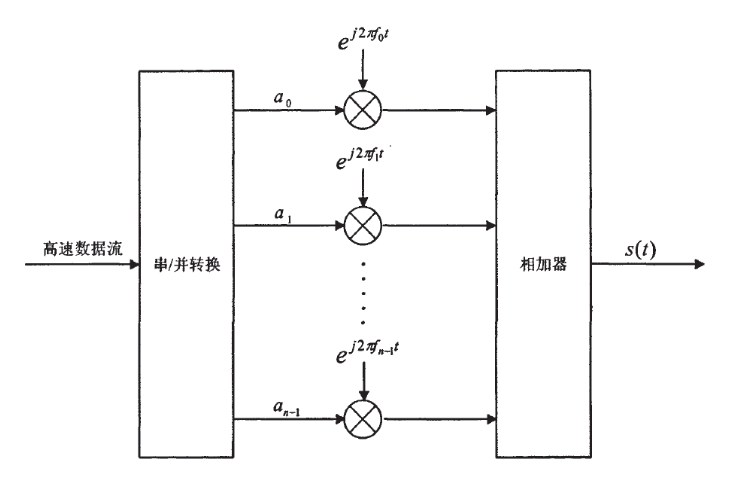图片是百度得来的，其中

a

0

a_0

就是式子中的

a

0

a_0

和

b

0

b_0

，框图中用了一个乘法器直接合成了

s

i

n

+

c

o

s

sin+cos

形式的子载波信号。
OFDM调制与IDFT：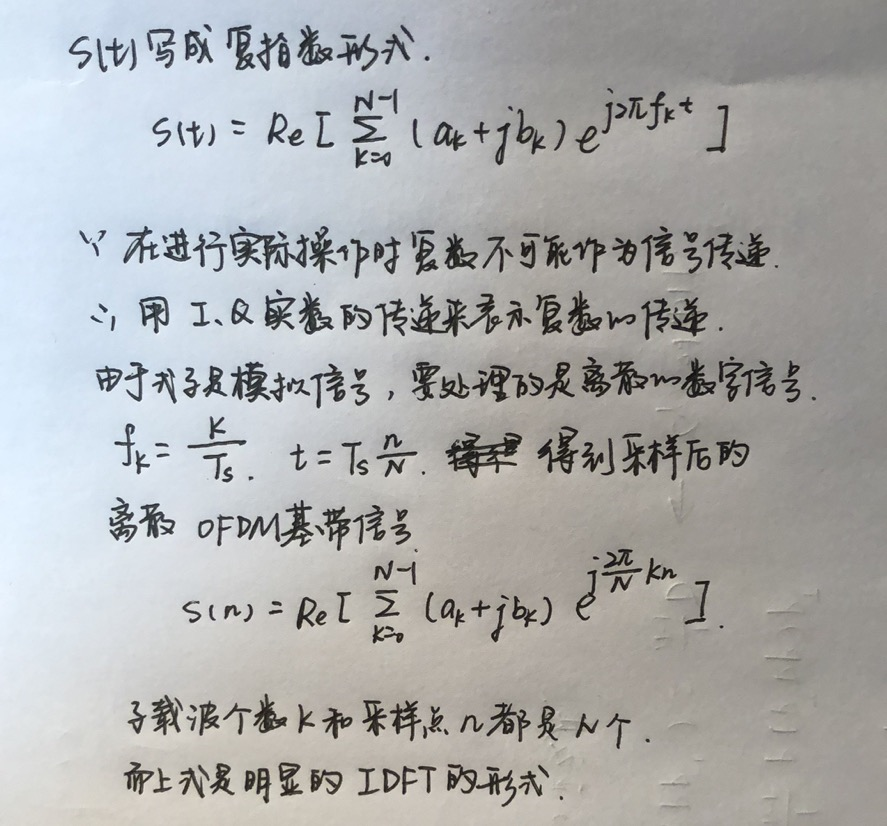展开全文• FPGA实现OFDM（1）-OFDM原理 失 踪 人 口 回 归 OFDM定义 fromwiki:调制是将传送资料对应于载波变化的动作，可以是载波的相位、频率、幅度、或是其组合。正交频分复用之基本观念为将一高速资料流程，分割成数个低速...fpga 数字通信 无线通信
• OFDM 基本原理 一个完整的 OFDM 系统原理 1 所示。OFDM 的基本思想是 将串行数据,并行地调制在多个正交的子载波上,这样可以降低每个 子载波的码元速率
• 序：既然已经下定决心弄懂OFDM，期末考前也为此准备了几天，如今期末考试结束之后，暑假到来前，想把这个问题好好总结一番，于是就有了如下一个小的系列，问题列表如下：好，这篇博文就讲原理图问题，可能不太完善，...
• 介绍不知怎么的碰(bei)巧(ju)和OFDM对上了。 这是一个大坑，很大又绕不过去的坑，不是三言两语能够解释清楚的，目测需要融合贯通理解数学的三角函数，傅里叶，通原的检波，调制，信道特征，信号处理的采样，电子元件...
• ## 给“小白”图示讲解OFDM的原理

万次阅读 多人点赞 2014-01-21 18:01:32
上面1.1-1.3的扩展，可如下所示： 七：时域上的OFDM系统 1.4 还有这一步吗？其实是有的。"小白"你可以先想想，想不到的话先往下看，因为这需要在频域中考量，所以我写在后面了。【也可参考】 将上述的时域...
• 上面1.1-1.3的扩展，可如下所示： 七：时域上的OFDM系统 1.4 还有这一步吗？其实是有的。"小白"你可以先想想，想不到的话先往下看，因为这需要在频域中考量，所以我写在后面了。【也可参考】 将上述的时域...
• OFDM时域削峰法的详细说明可参考：https://www.cnblogs.com/achangchang/p/11037498.html 转载于:https://www.cnblogs.com/achangchang/p/11027839.html
• 正交频分复用OFDM（OrthogonalFrequencyDivisionMultiplex)是一种多载波调制方式，通过减小和消除码间串扰的影响来克服信道的频率选择性衰落。它的基本原理是将信号分割为N个子信号，然后用N个子信号分别调制N个相互...
• OFDM的基本原理是将高速的数据流分解为多路并行的低速数据流，在多个载波上同时进行传输。对于低速并行的子载波而言，由于符号周期展宽，多径效应造成的时延扩展相对变小。当每个OFDM符号中插入一定的保护时间后，码...
• 学习的内容主要关于OFDM技术，在这里会介绍OFDM技术的原理，用到了载波频率调制的知识进行原理分析。 一、占空比为40%的矩形波频谱分析 矩形波的产生：利用zero函数建立一个长度为100的信号，使40%内的信号置一，...MATLAB 频率调制
• 信道的特征向量4.OFDM的优缺点4.1优点4.2缺点 1.基本的接收模型 yn=∑t=0N−1hn(t)⋅xn−t+nny_n=\sum_{t=0}^{N-1}h_n(t)\cdot x_{n-t}+n_nyn​=t=0∑N−1​hn​(t)⋅xn−t​+nn​又我们有 xn=1N∑k=1KXkej2πnkN,...
• ## 理解OFDM技术原理

千次阅读 多人点赞 2020-06-21 15:25:23
• ## 正交频分复用(OFDM)原理及实现

万次阅读 多人点赞 2019-04-01 17:01:42
1.OFDM原理 1.1时域正交与基带信号 1.2频域正交与频率间隔Δf 2.基带信号的调制框图 3.OFDM调制与IDFT 4.串行比特流怎么进行IDFT 1.OFDM原理 1.1时域正交与基带信号 OFDM的核心就是利用各个子载波的正交...正交频分复用
• 时间回到07年底，4G方兴之时，同桌隔壁的隔壁"小白"同学说看不太明白OFDMA的原理，让我讲解一下。我一向对自己的技术水平、逻辑思考能力和表达技巧还是蛮有自信的，因此轻笑一声就答应了。半小时后，在尝试了从时域...OFDM
• ## OFDM基本原理2

千次阅读 2014-07-31 11:36:44
原文地址：OFDM基本原理2作者：玄子直接产生OFDM信号   第三个等号相当于把高频信号s(t)分解为低频信号与载波fc。N个载波只使用2k+1个载波传送信号（包括1路直流）。   ICI   接收端同步做不好，就会出现干扰...
• ## OFDM信号频谱图

千次阅读 2018-10-26 14:03:54
OFDM原理网上讲解的很多，但是真的画频谱，连个基本的程序都没有找到。下面附上自己写的MATLAB clc clear all; j=sqrt(-1); B=100e6;%100Mhz N=64;%子载波数number of subcurries T=(N+1)/B;%码元持续时间 ...MATLAB
• 章节一：时域上的OFDM OFDM的"O"代表着"正交"，那么就先说说正交吧。 首先说说最简单的情况，sin(t)和sin(2t)是正交的【证明：sin(t)·sin(2t)在区间[0,2π]上的积分为0】，而正弦函数又是波的最直观描述，因此我们...
• OFDM是一种特殊的多载波传输方案，它可以被看做是一种调制技术，也可以被当作一种复用技术。多载波传输把数据流分解成若干子比特流，这样每个字数据流将具有低比特率，从而降低速率符号并行发送的传输系统。它也是对...
• ## OFDM基本原理学习1

千次阅读 2014-07-31 11:36:41
原文地址：OFDM基本原理学习1作者：玄子OFDM Basic Idea:   Split the high data-rate stream into a number of subsrteams that are transmitted in parallel over N subchannels.（Block transmission） N的选择...
• 章节一：时域上的OFDM 　OFDM的"O"代表着"正交"，那么就先说说正交吧。 　首先说说最简单的情况，sin(t)和sin(2t)是正交的【证明：sin(t)·sin(2t)在区间[0,2π]上的积分为0】，而正弦函数又......